# R S Aggarwal Solutions for Class 11 Maths Chapter 9- Combinations

R S Aggarwal Solutions are useful study materials for students as it helps them in scoring great marks in the examination. The R S Aggarwal Solutions given in this page contains detailed step-by-step explanations to all the problems that come under Chapter 9, Combinations, of the Class 11 R S Aggarwal Textbook.

These solutions are prepared by subject matter experts at BYJU’S, explaining the method of solving problems, right from the basic step. By understanding the concepts used in R S Aggarwal Solutions for Class 11 Maths, students will be able to clear all their doubts related to the chapter Combination.

### Download PDF of R S Aggarwal Solutions for Class 11 Maths Chapter 9- Combinations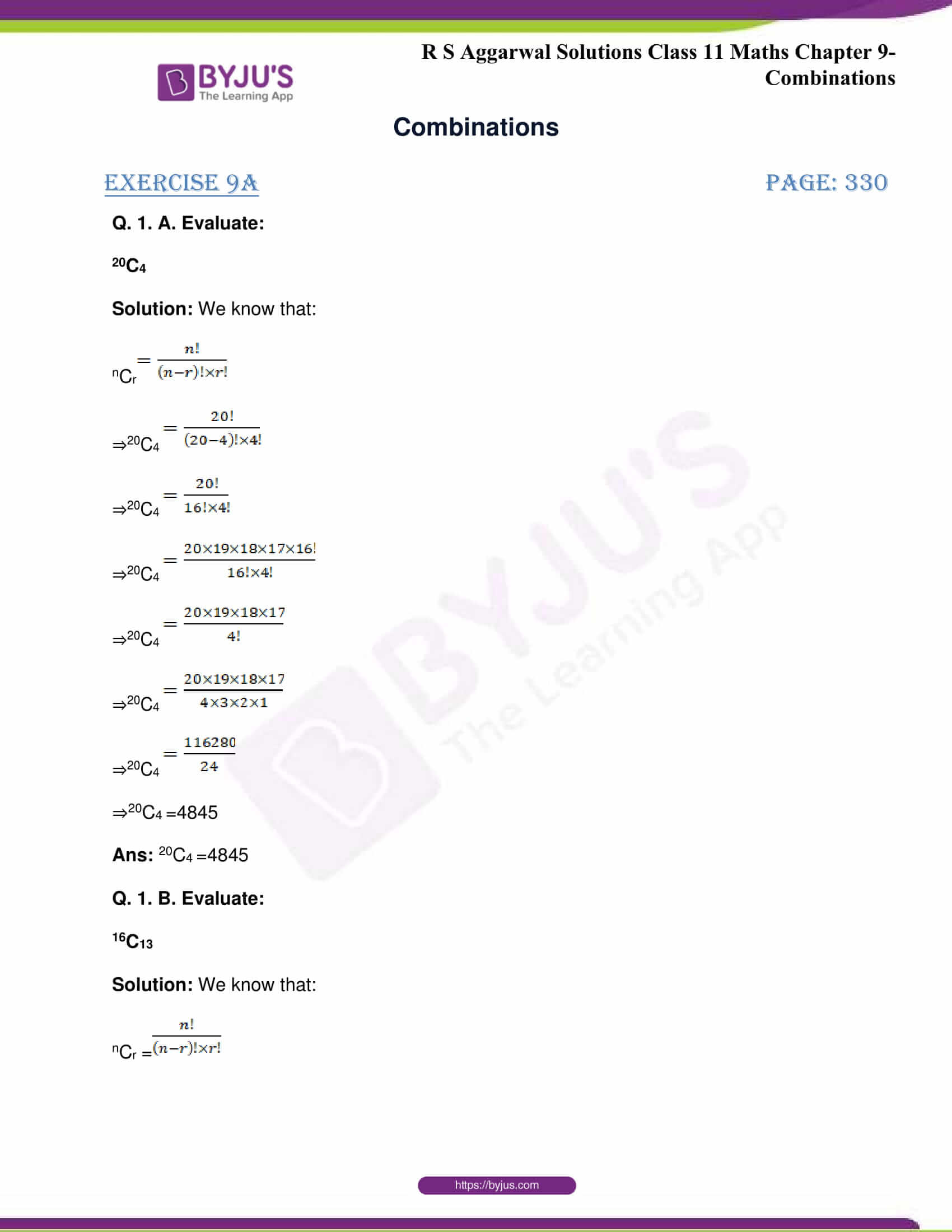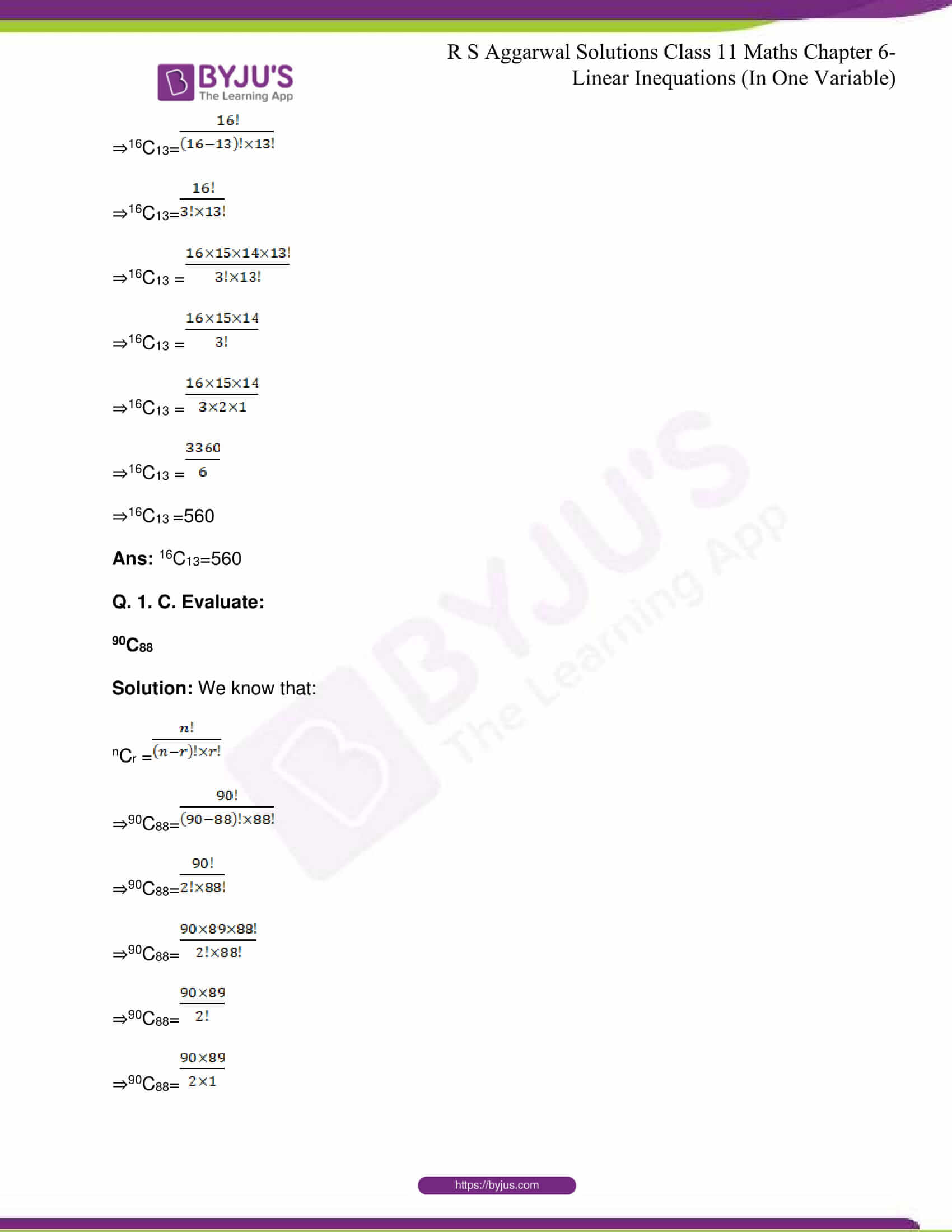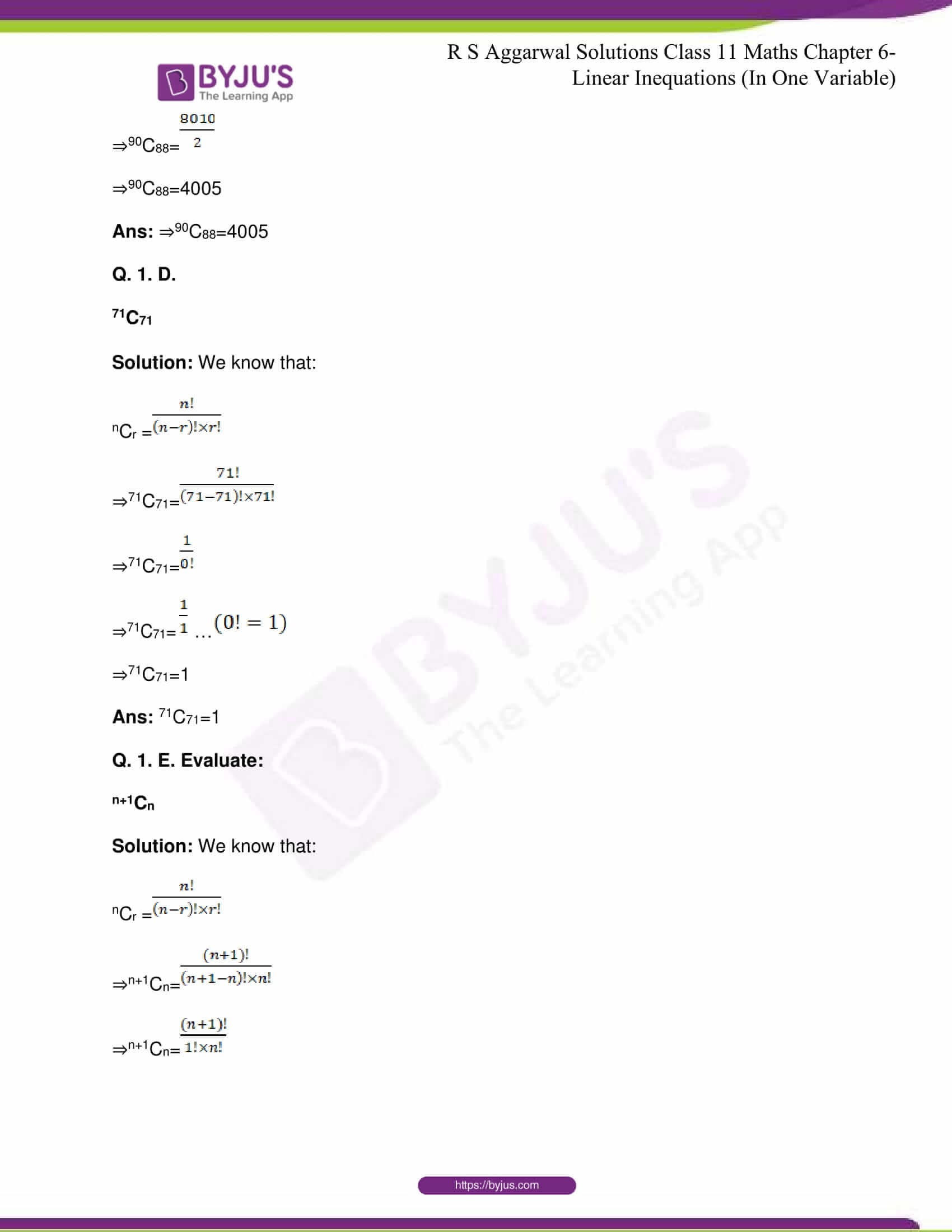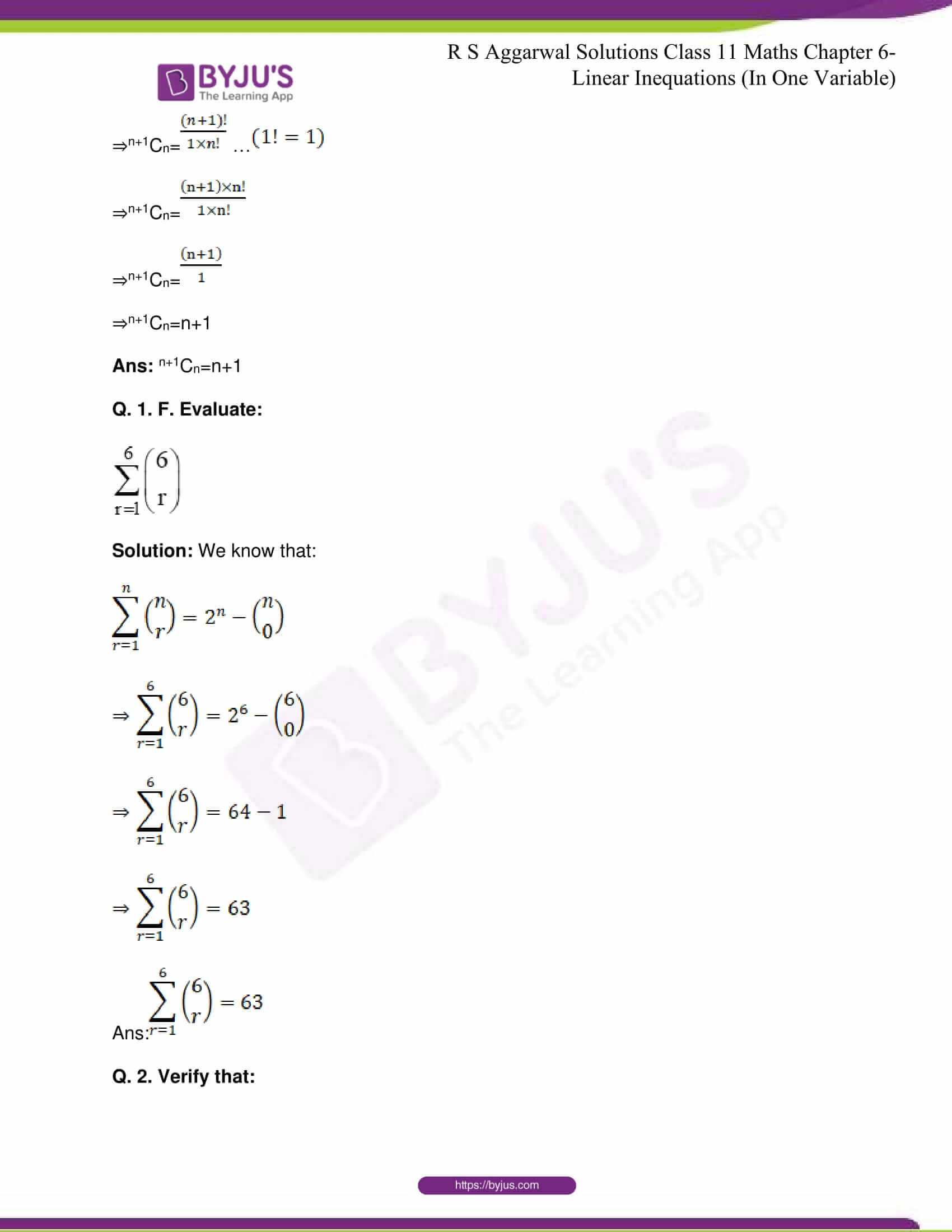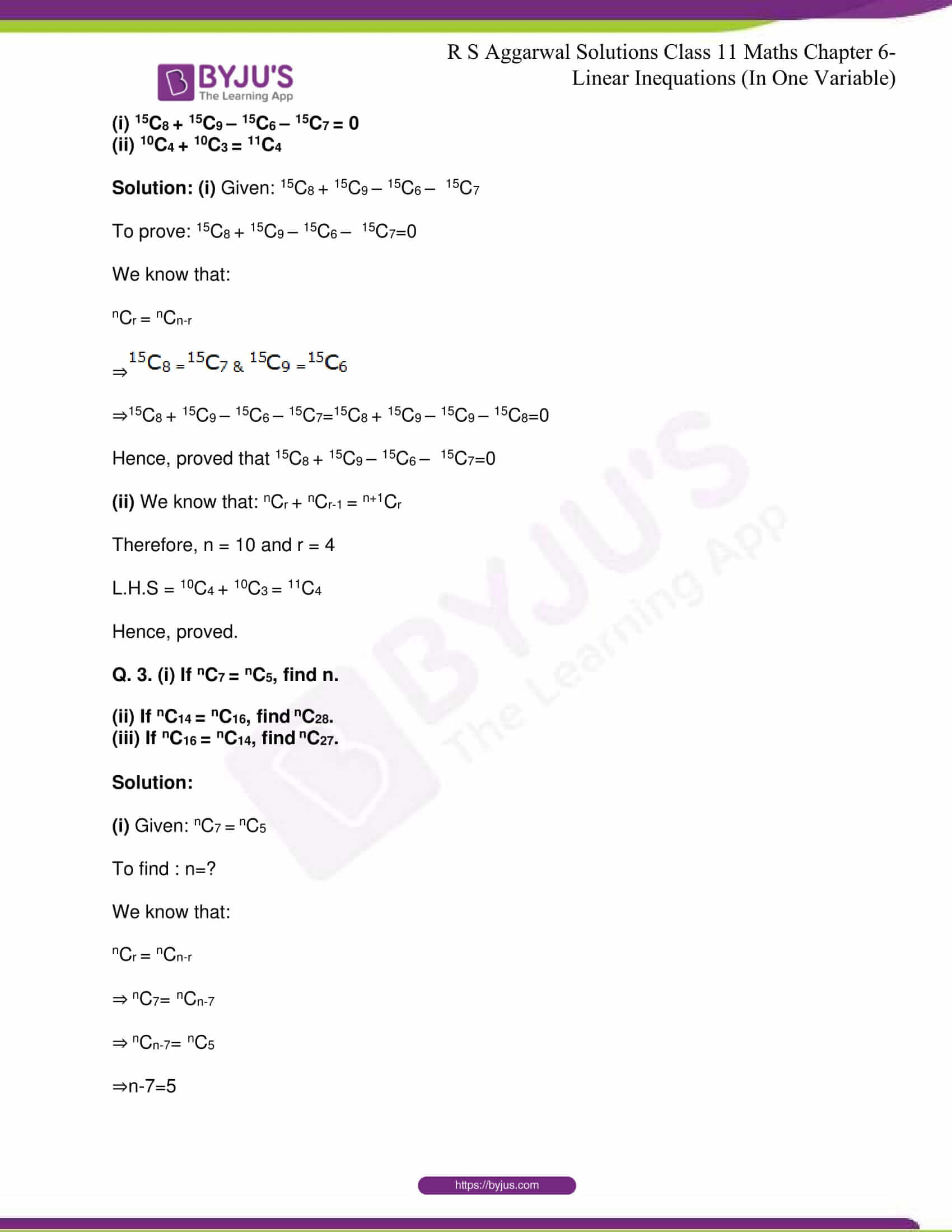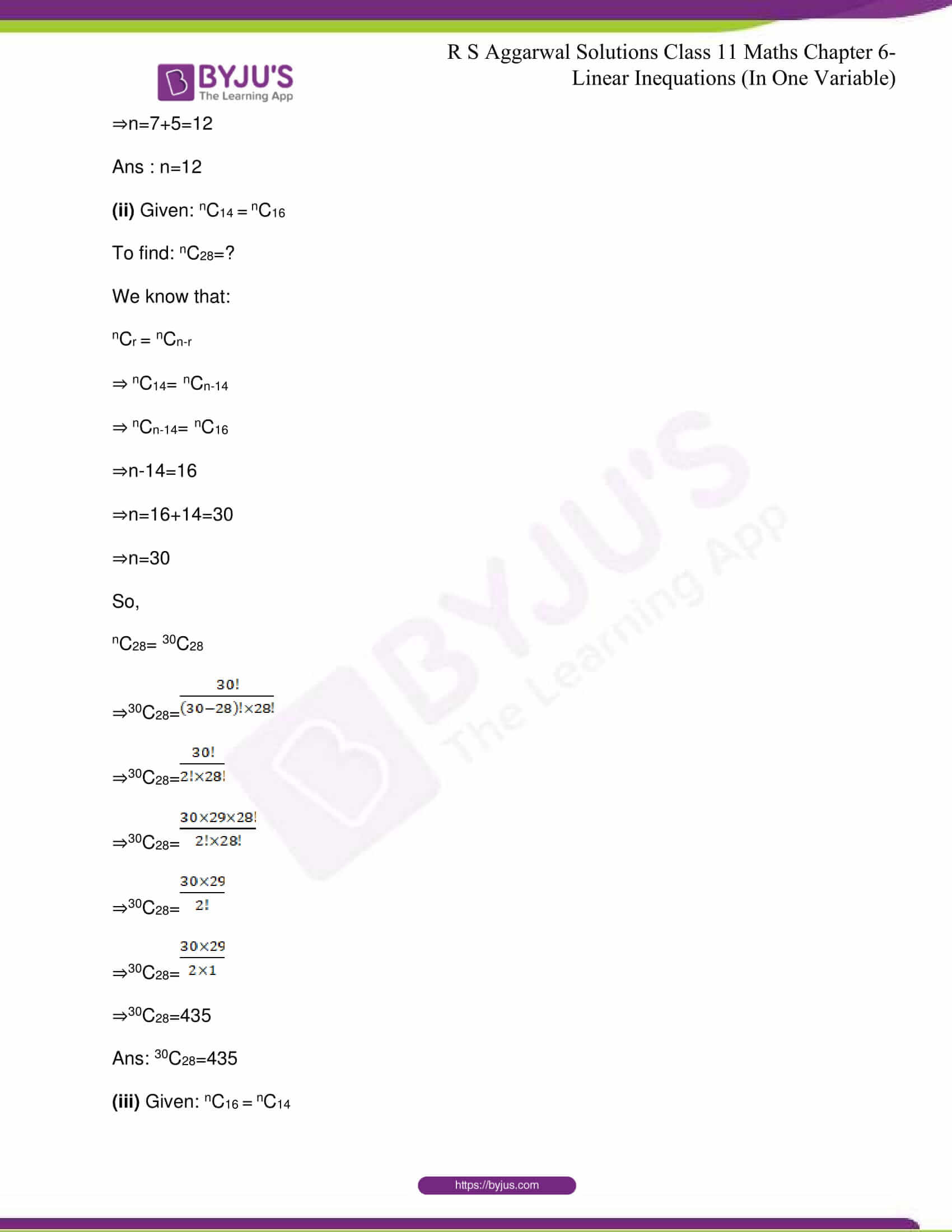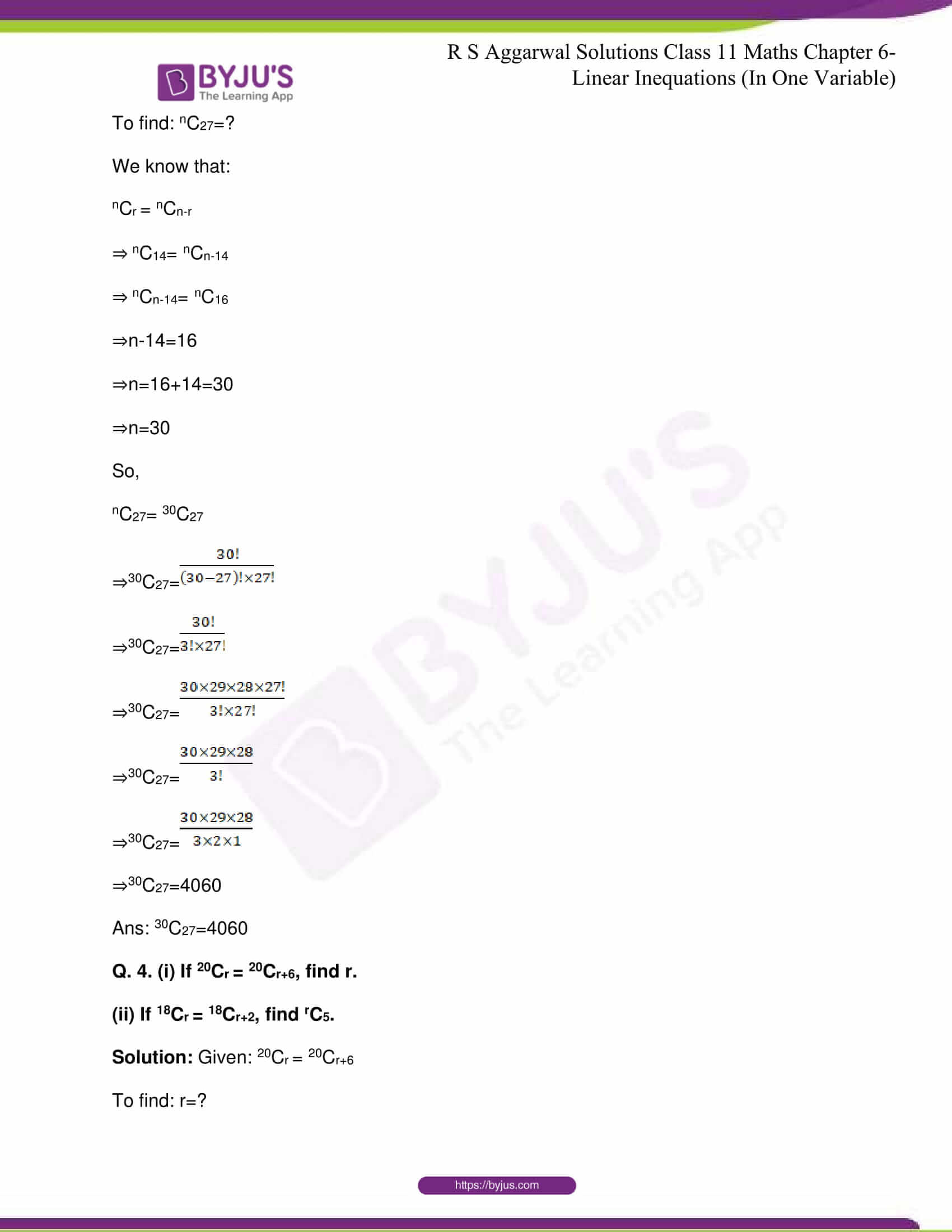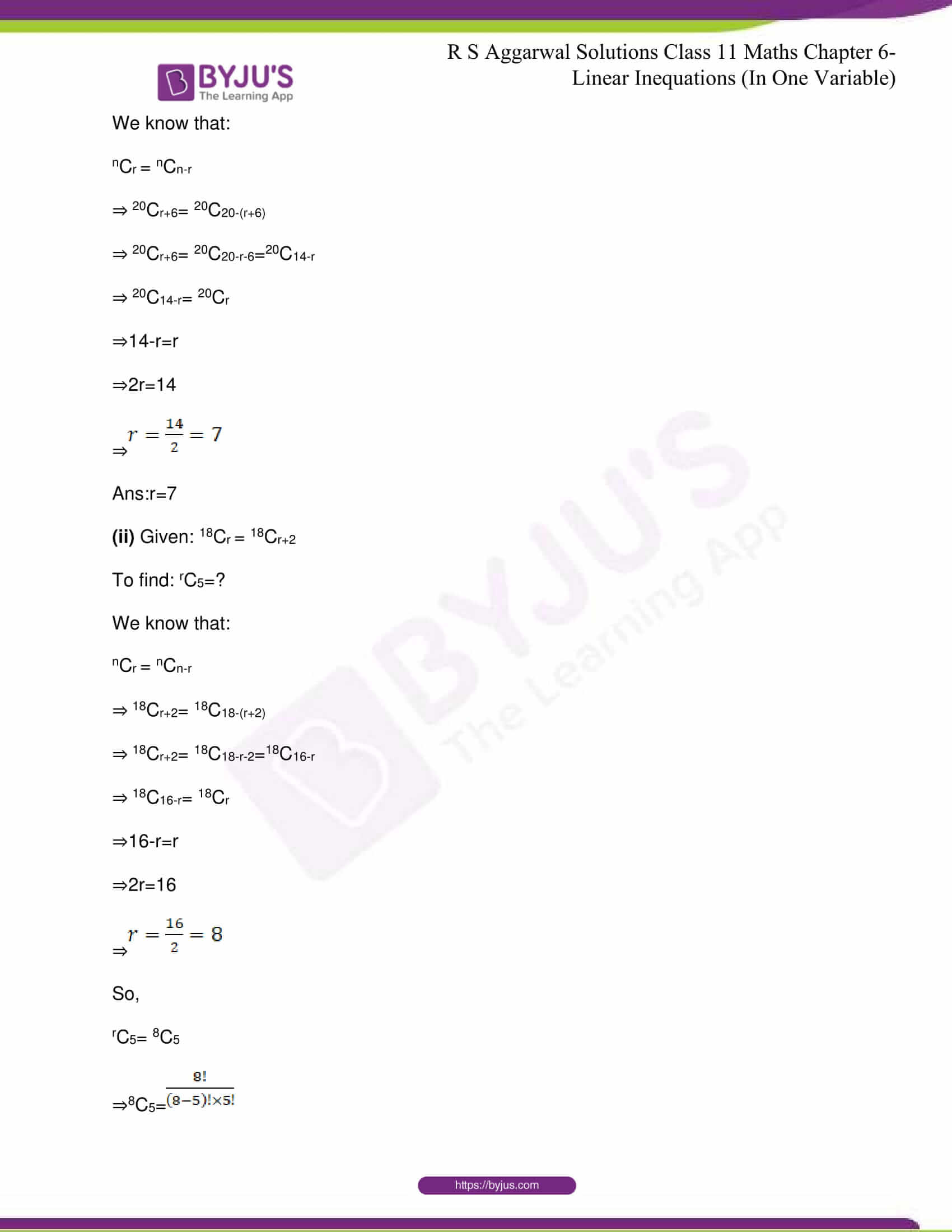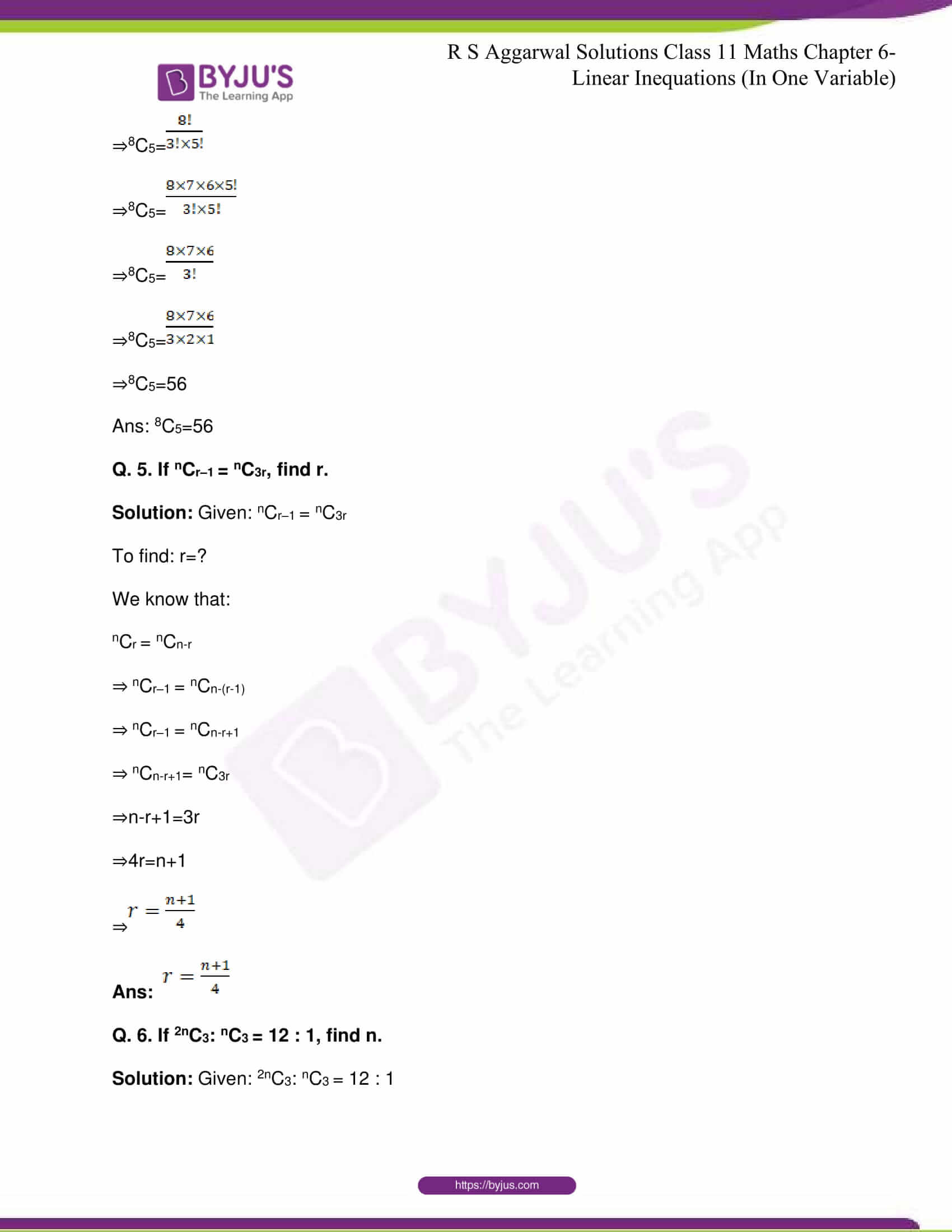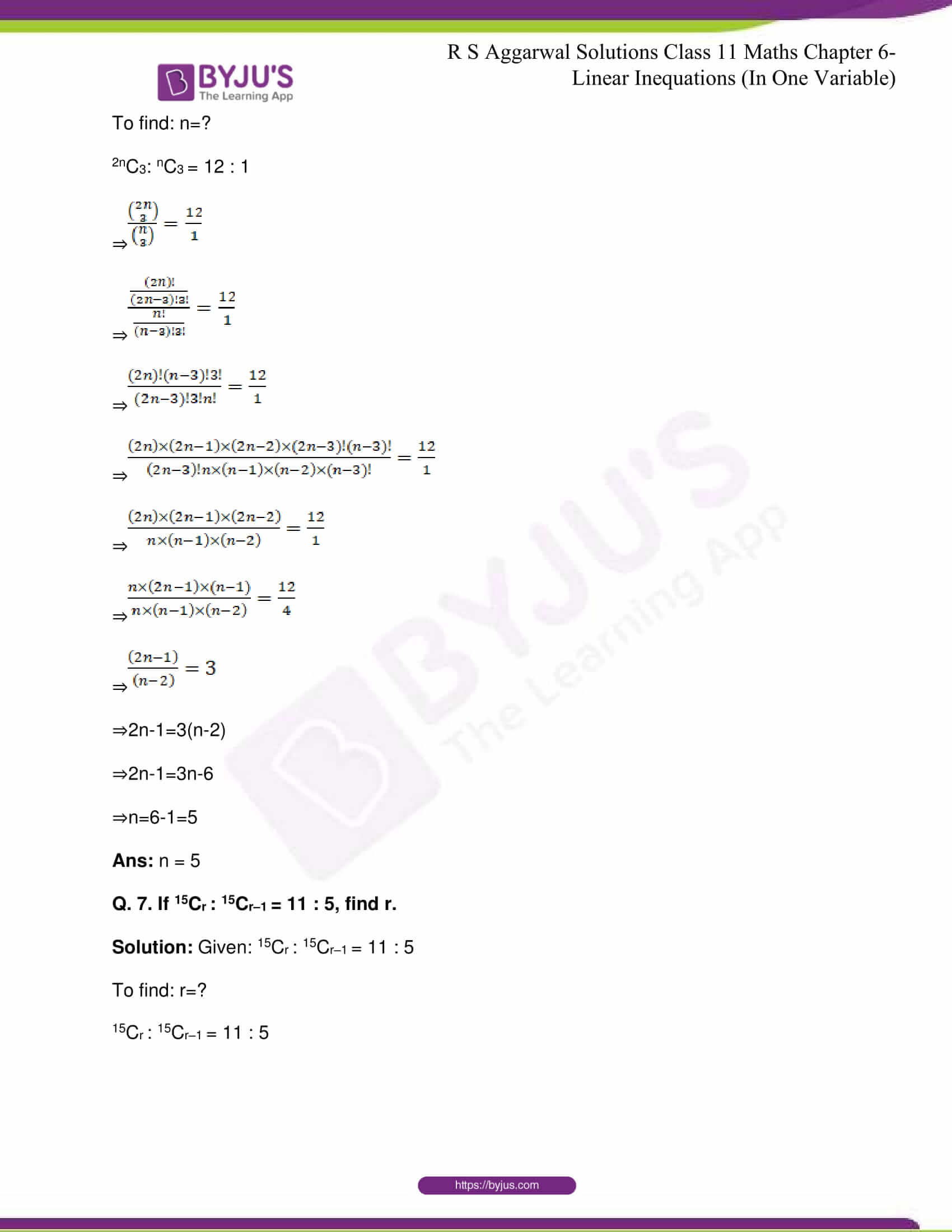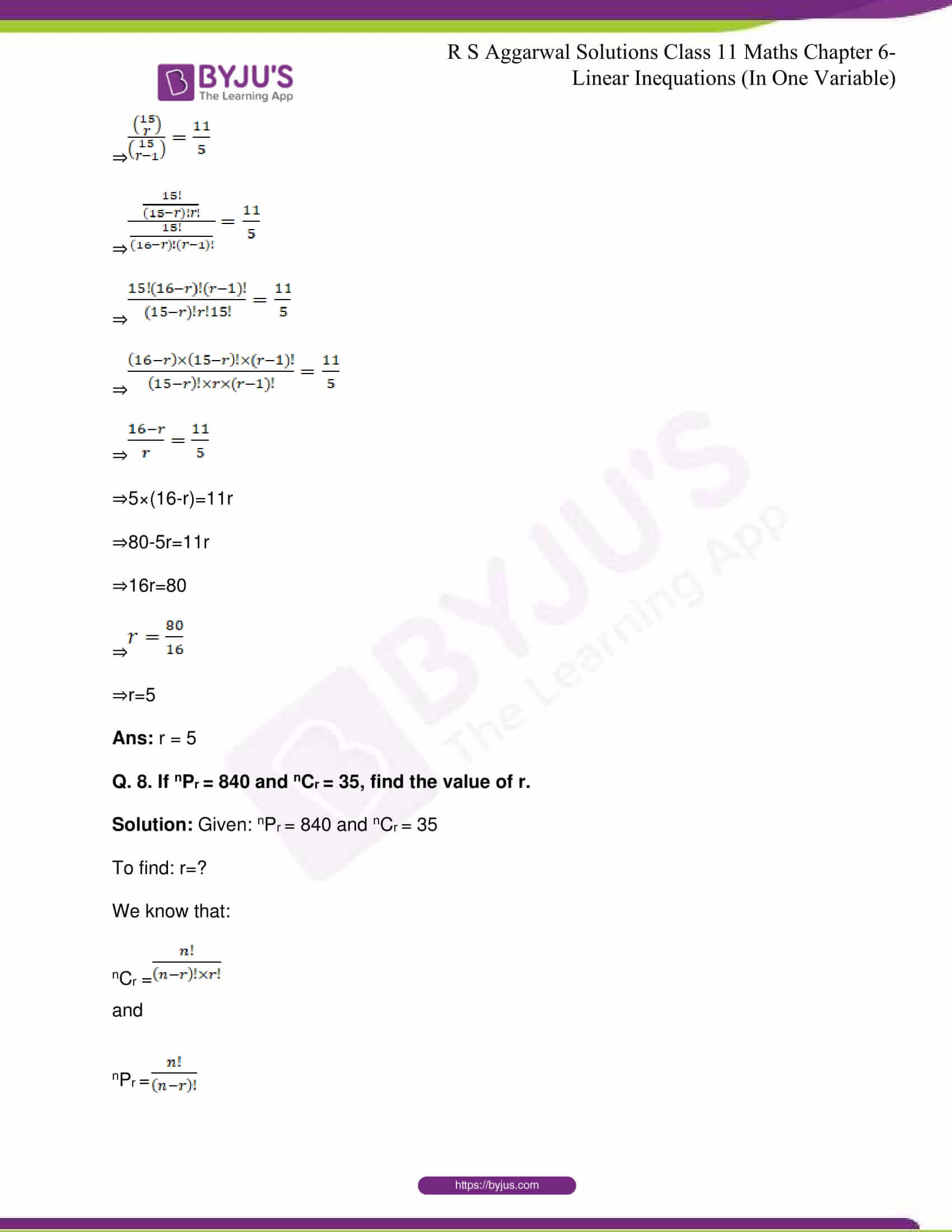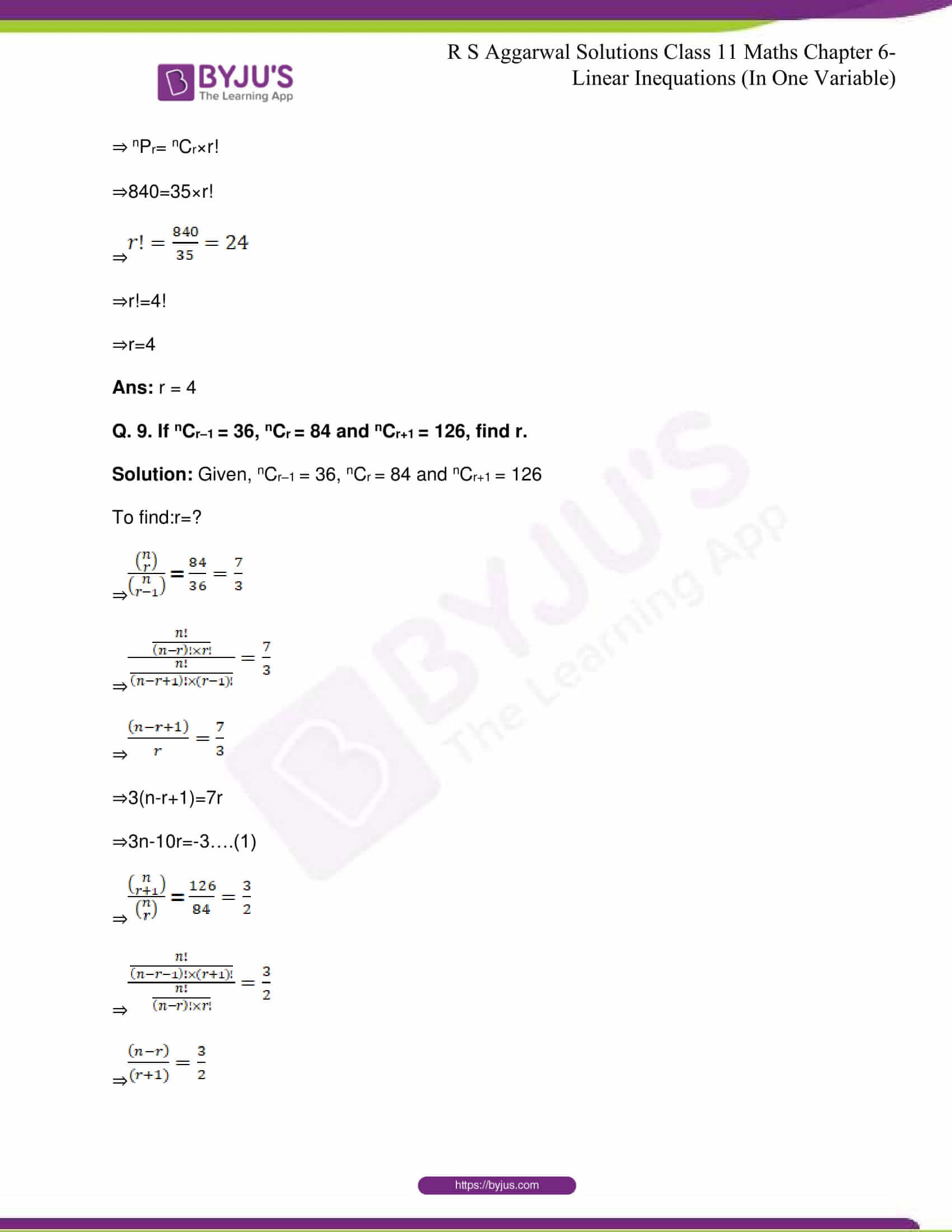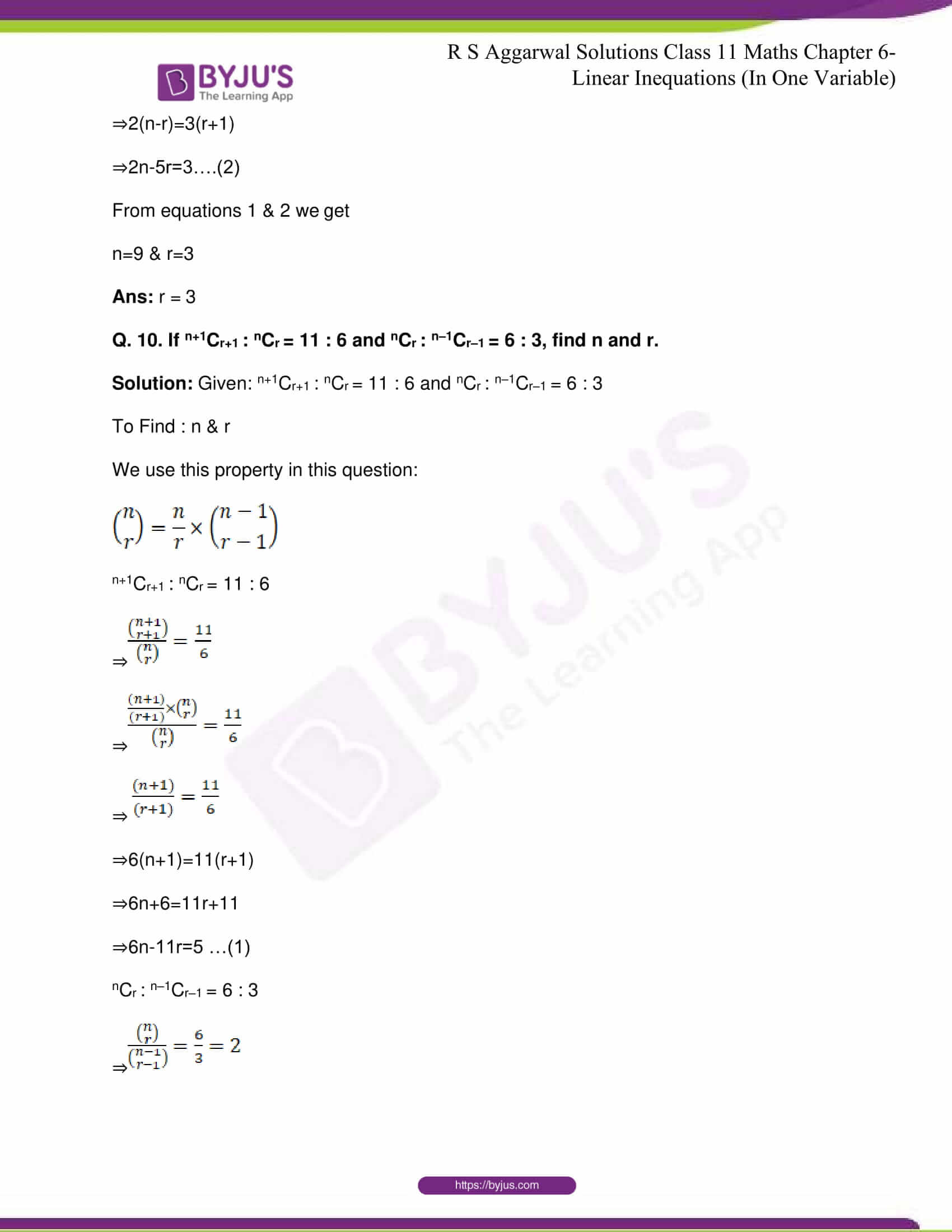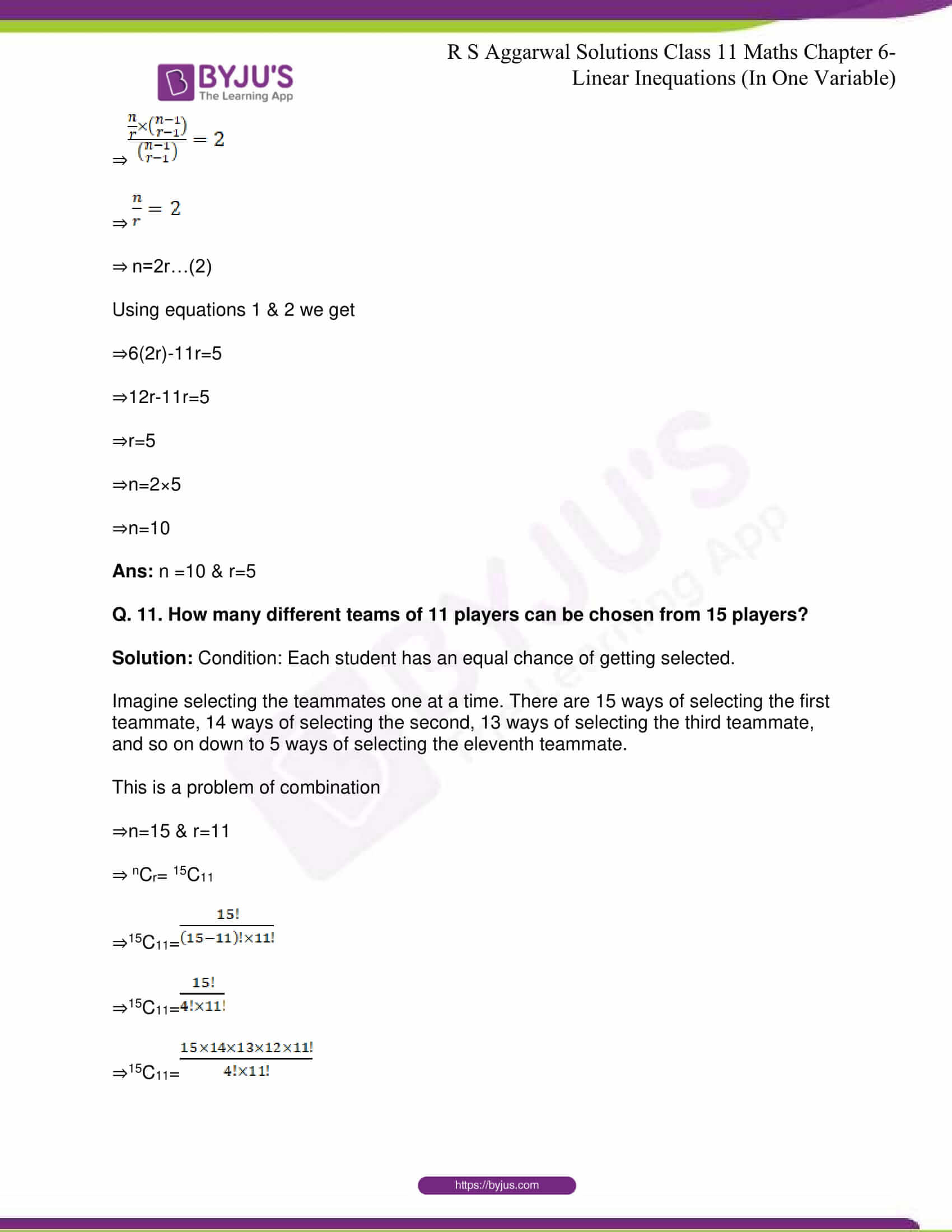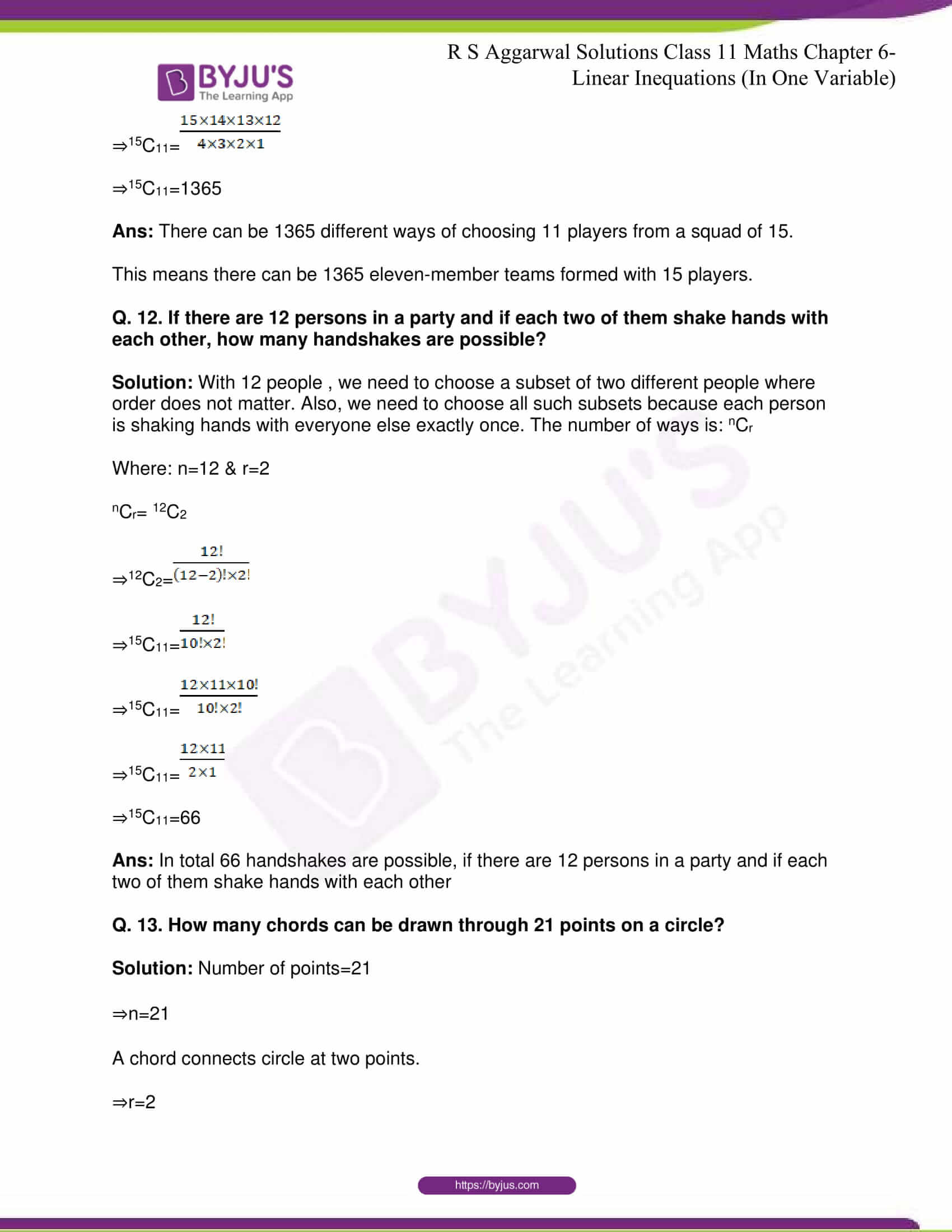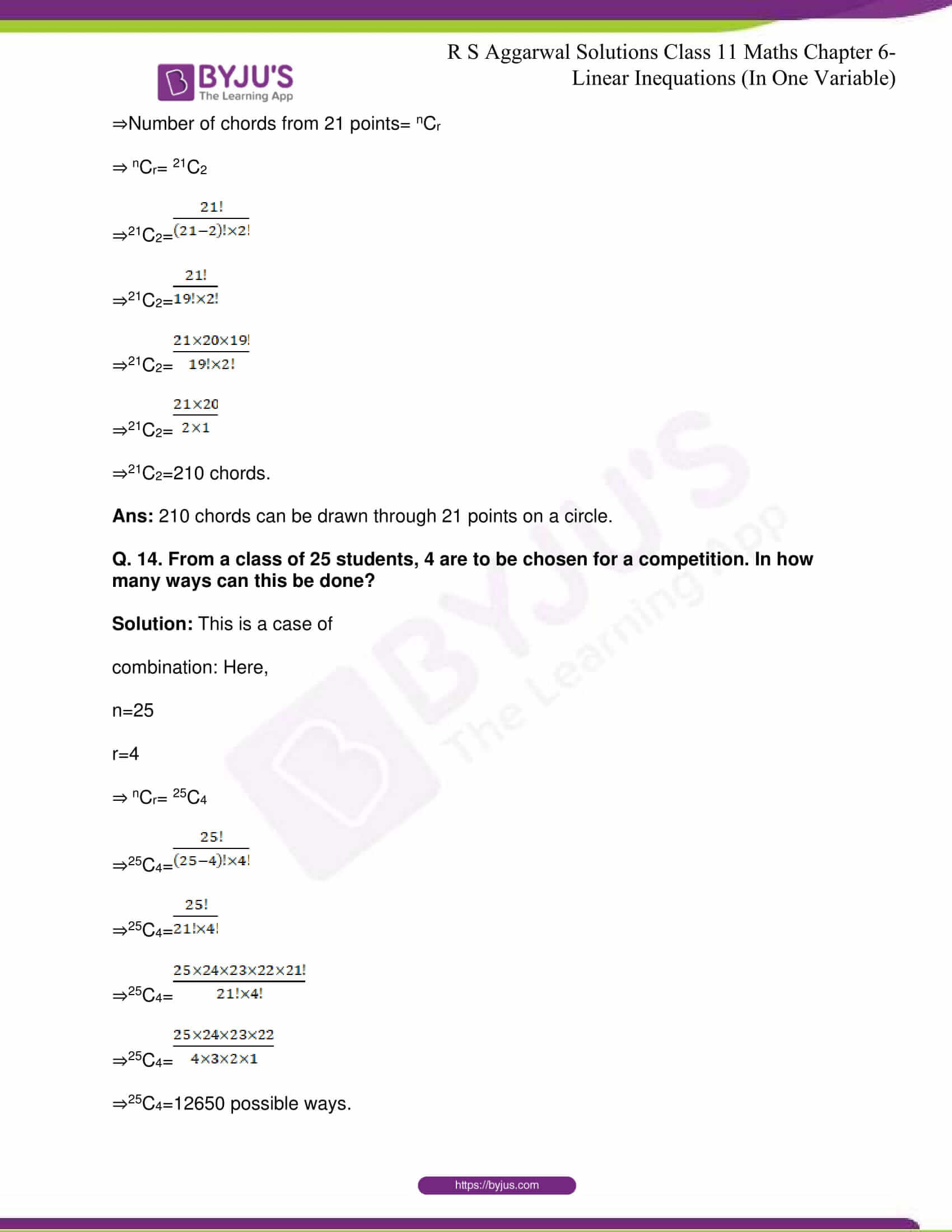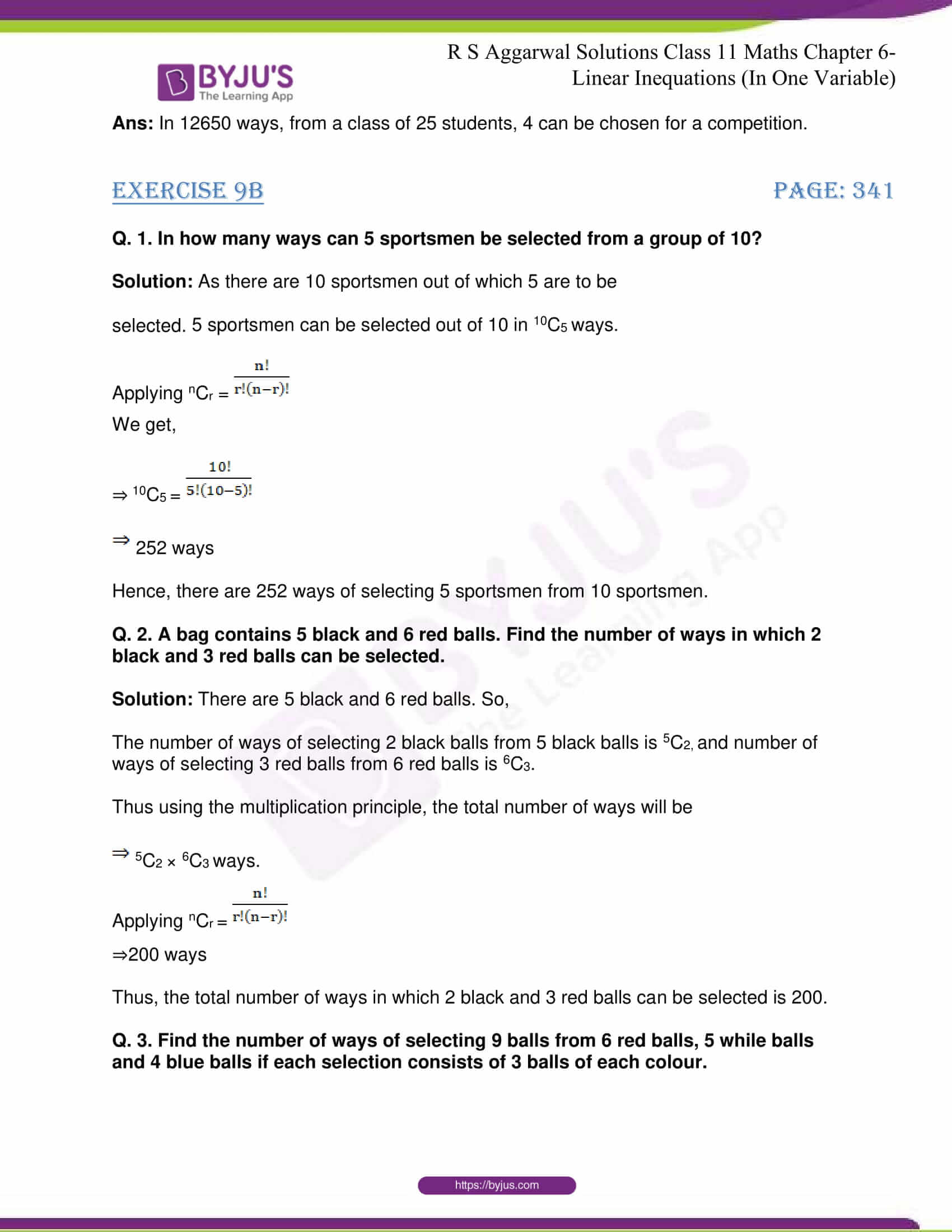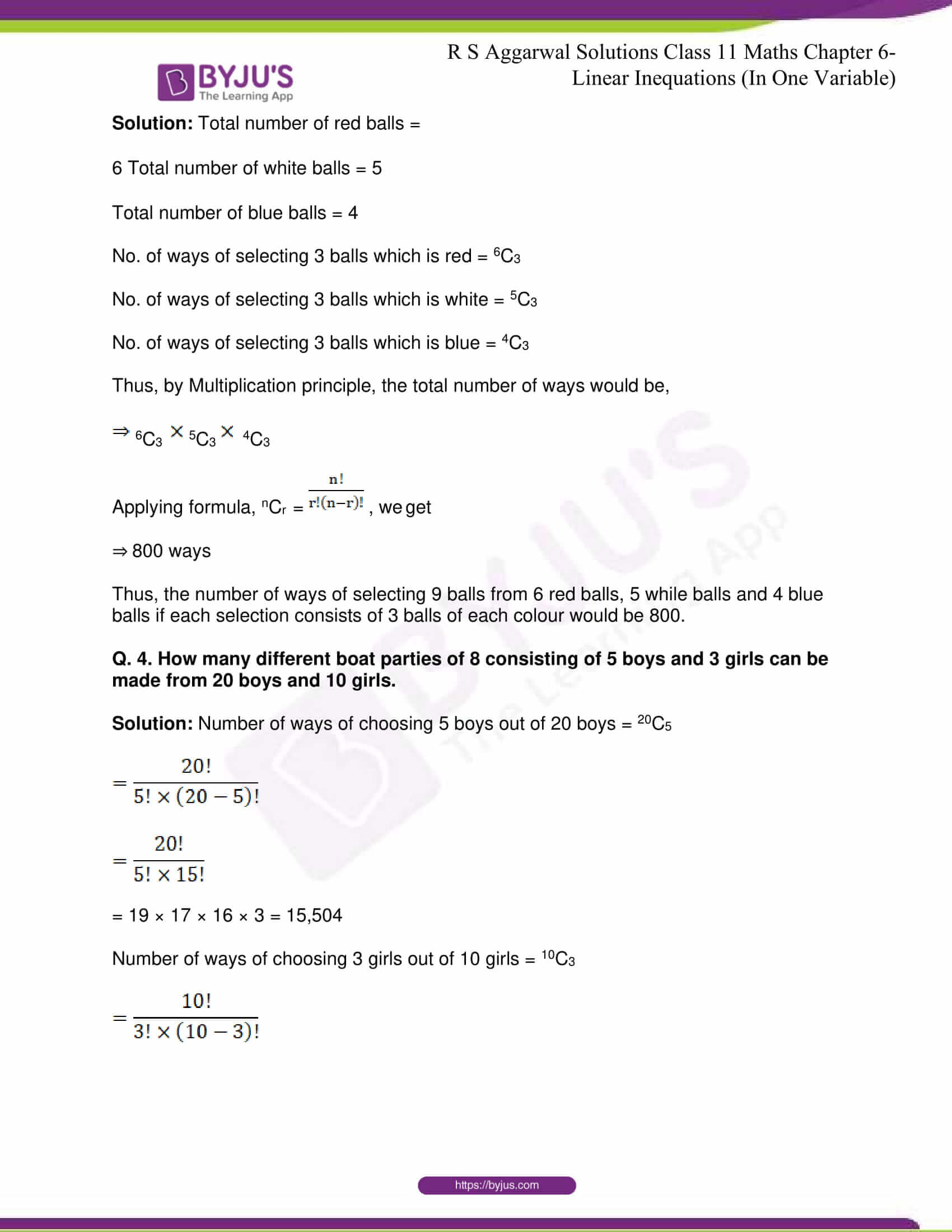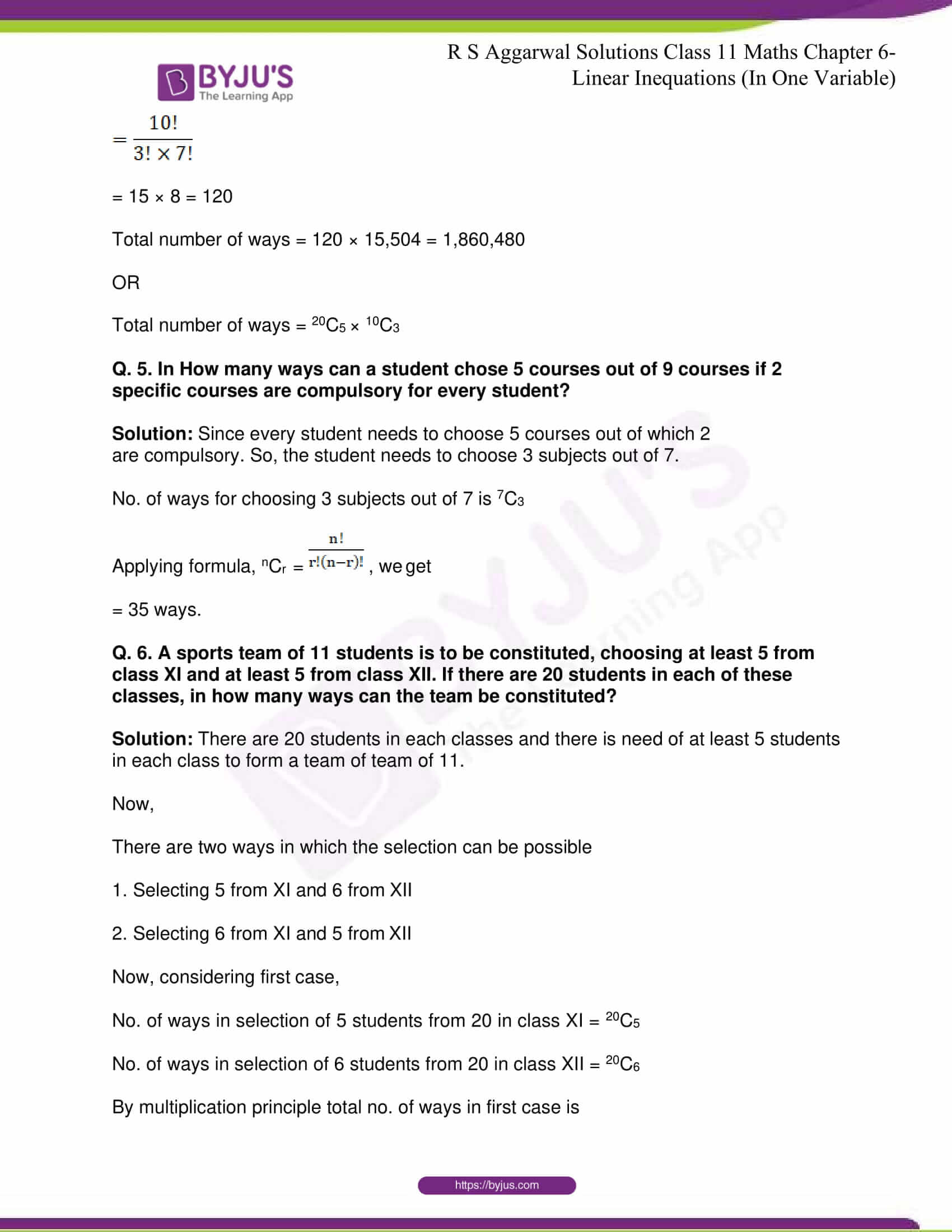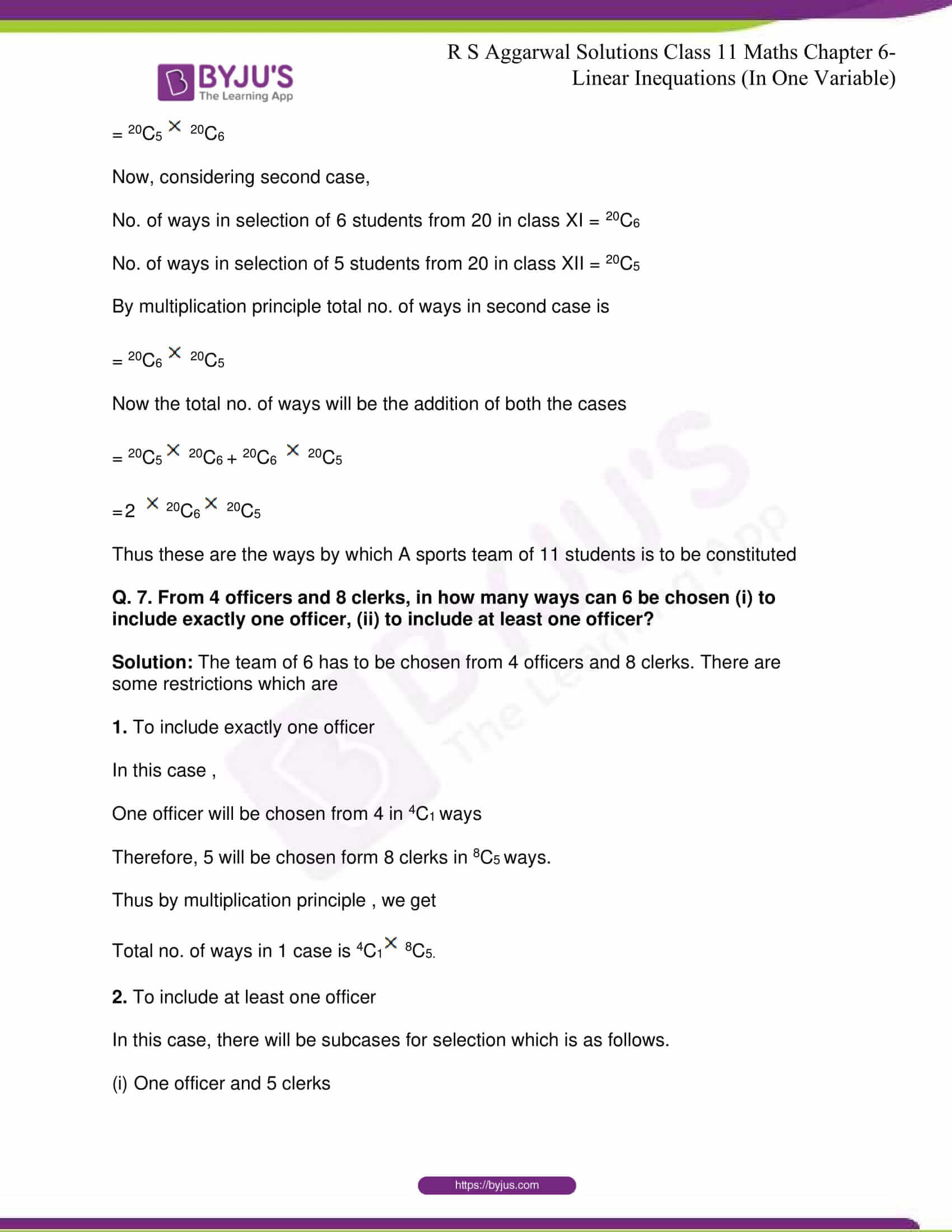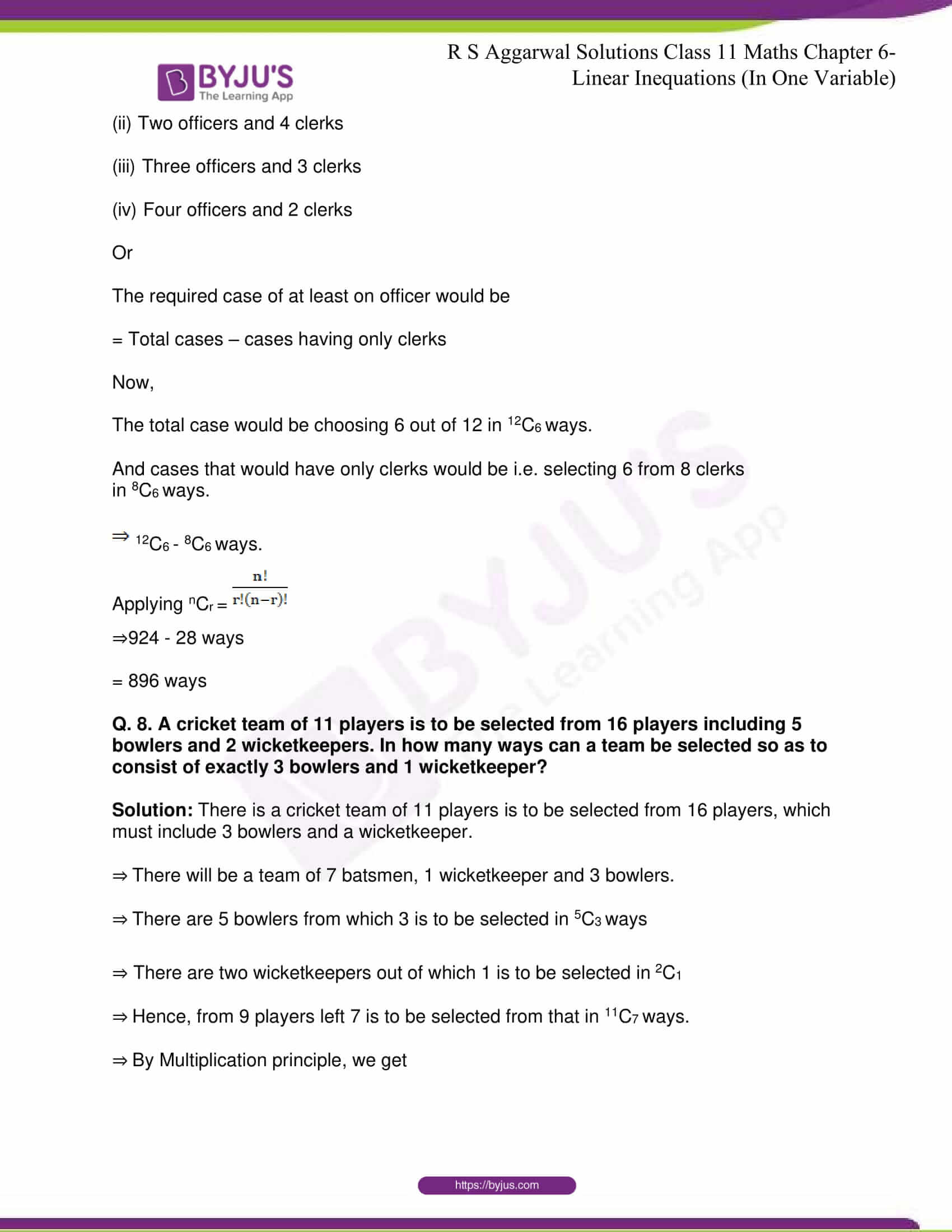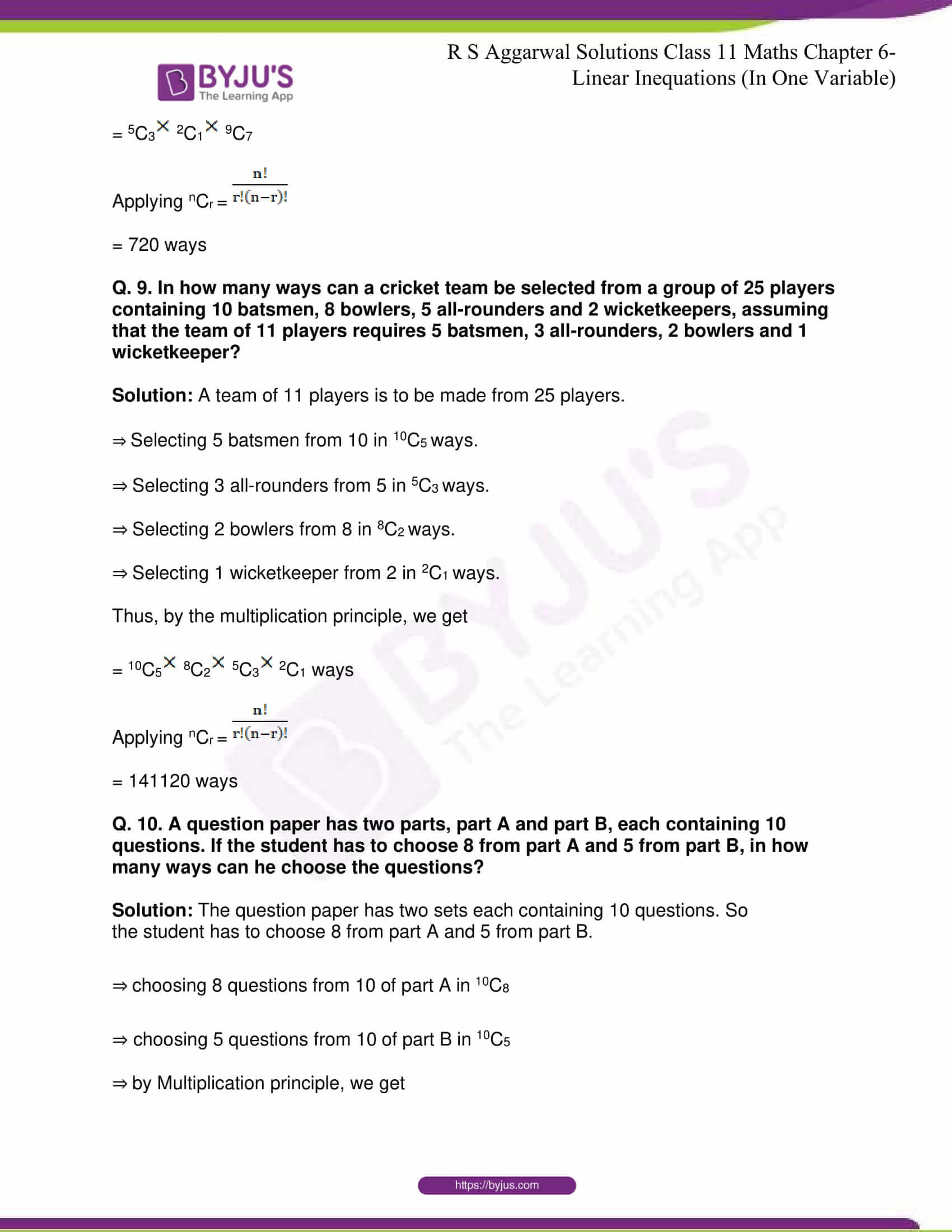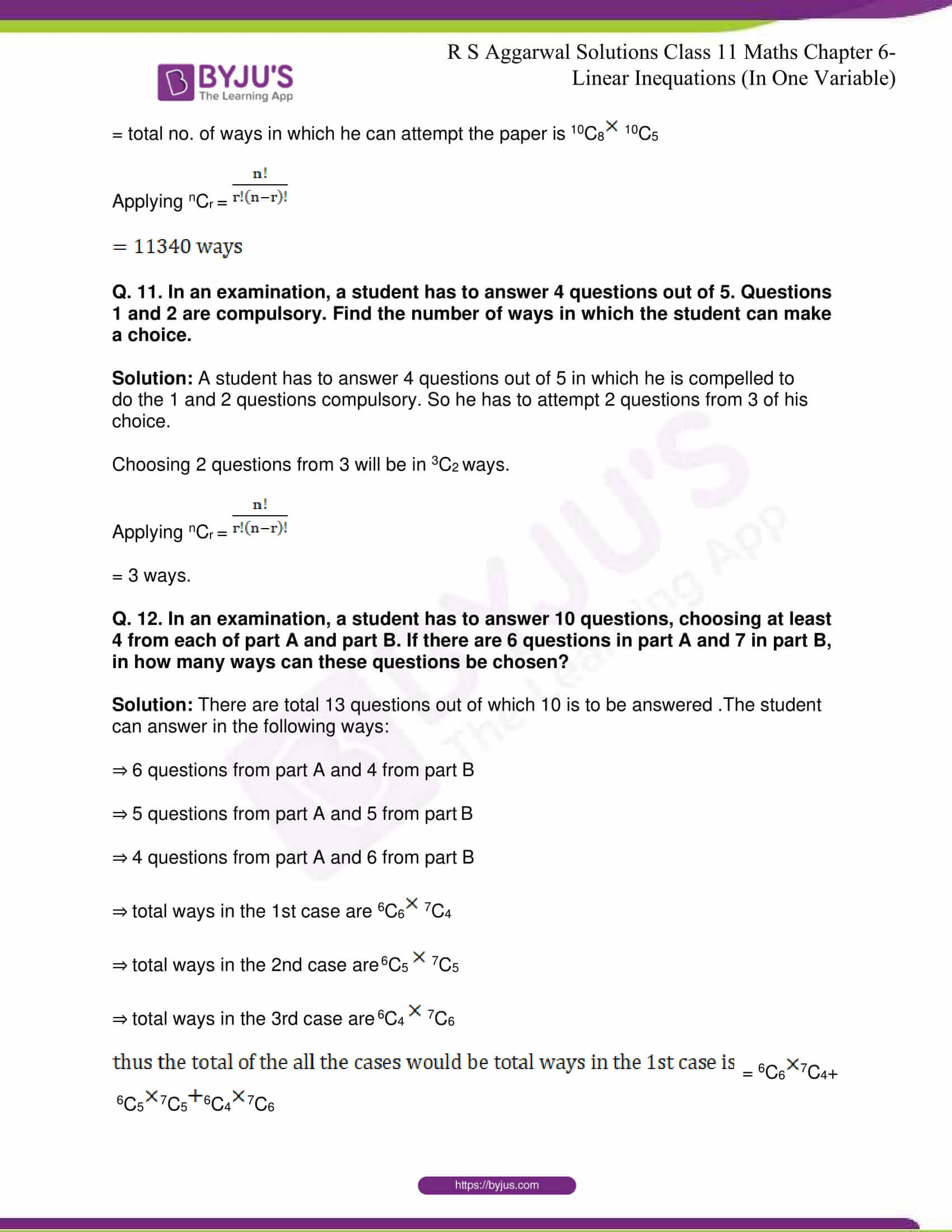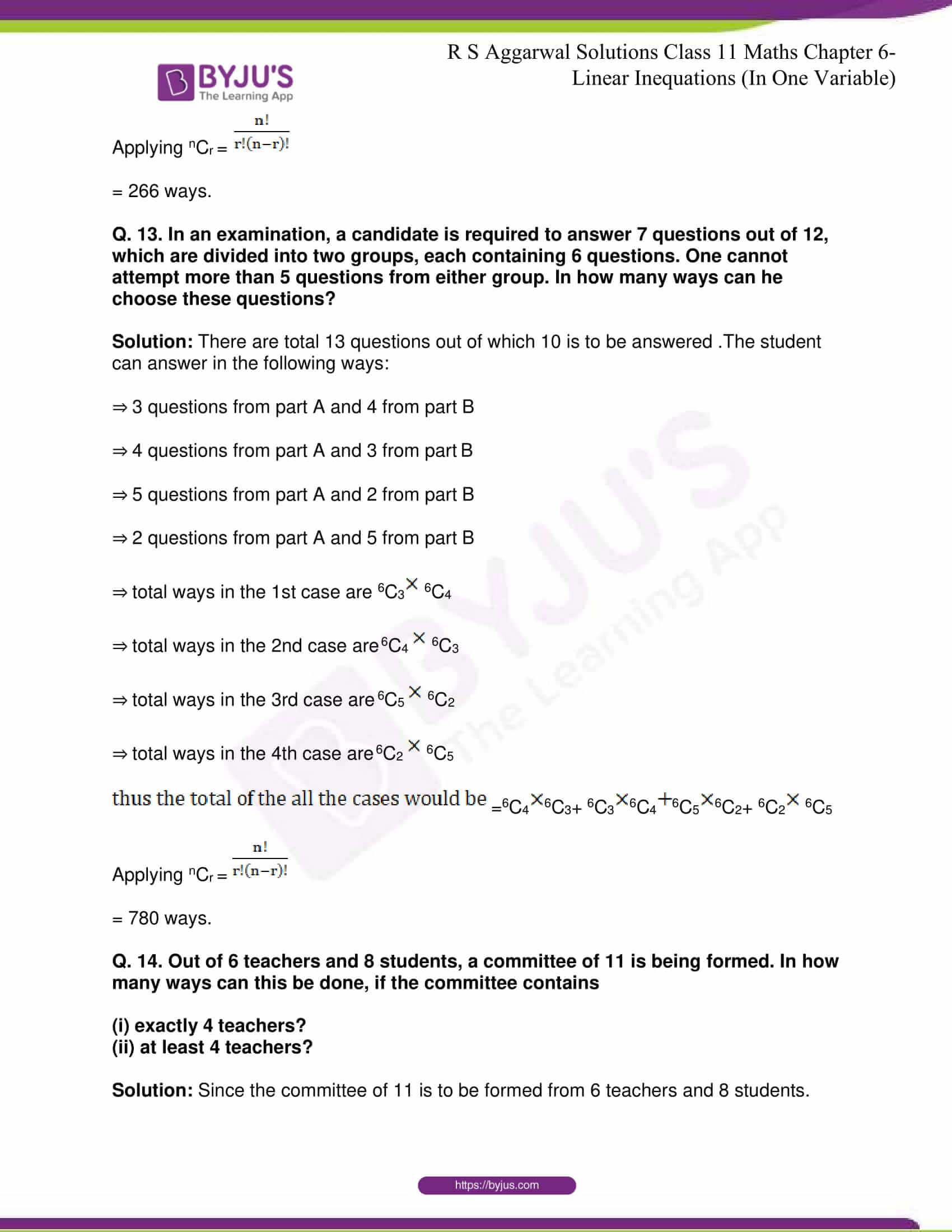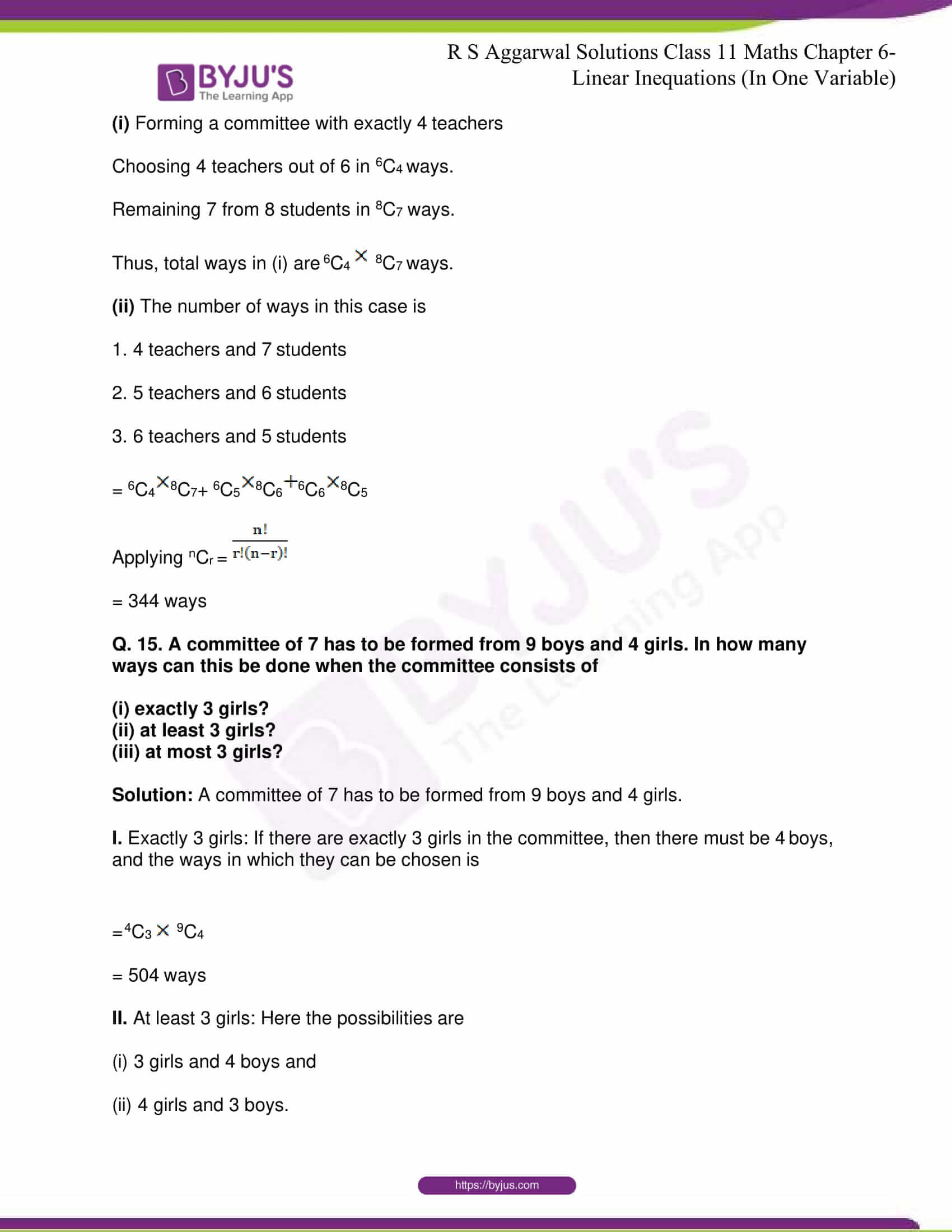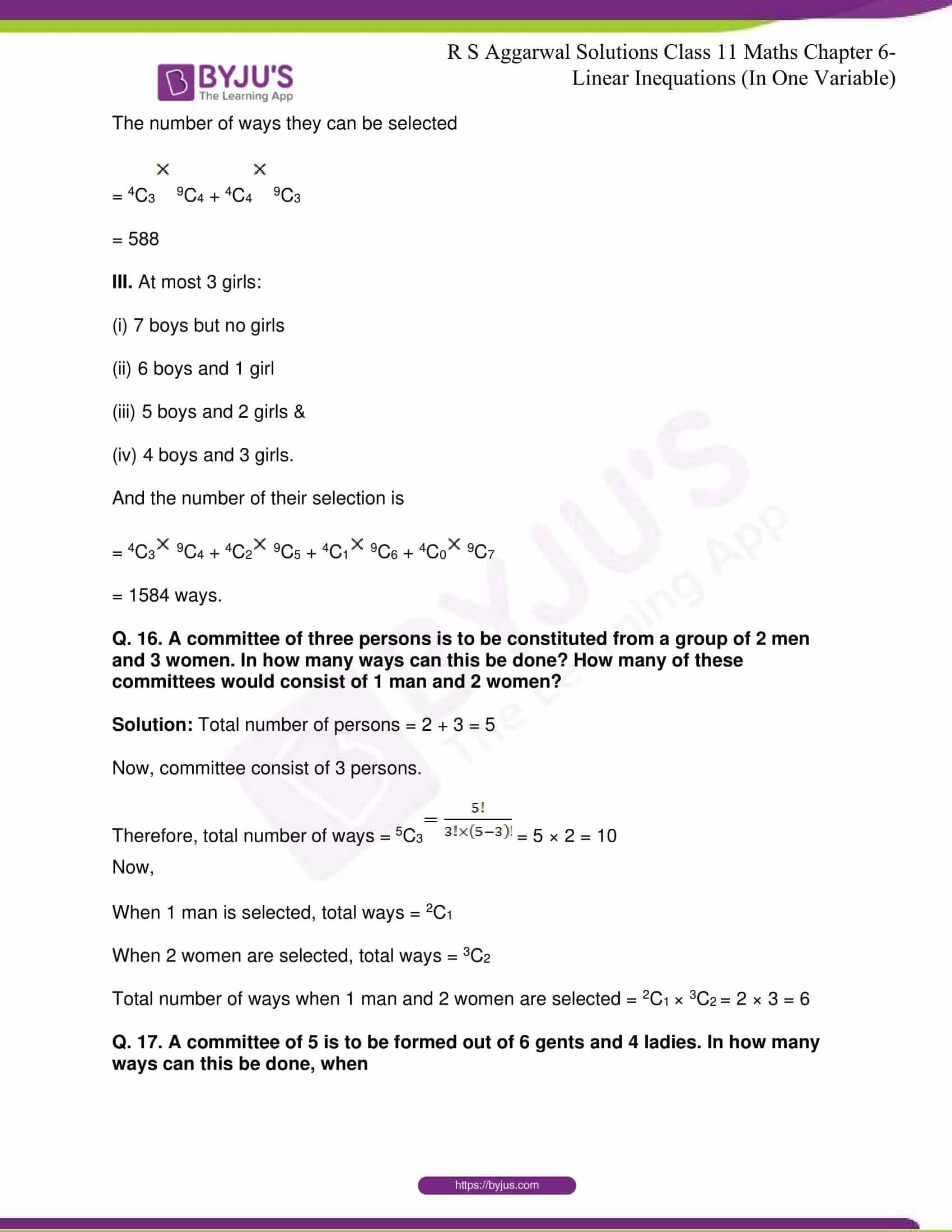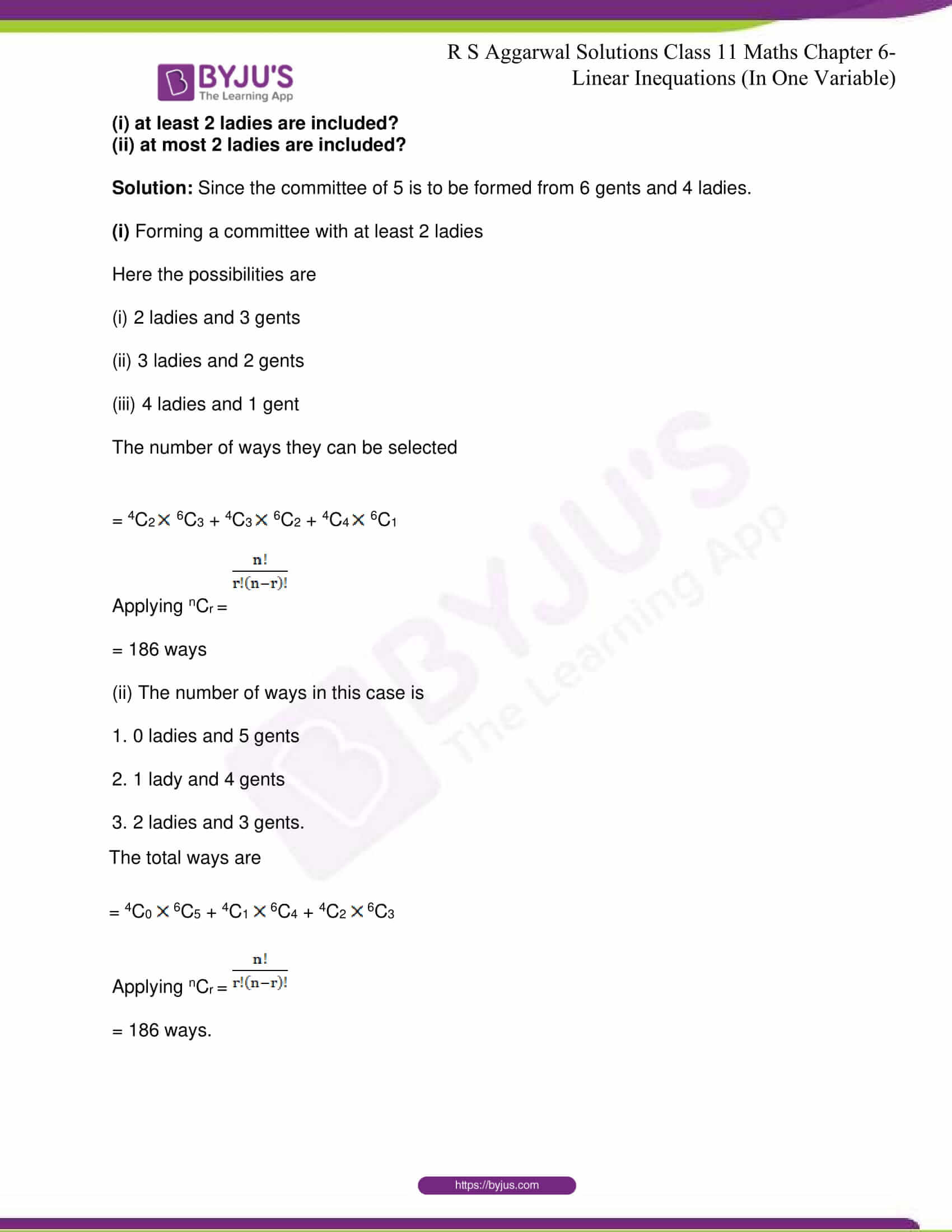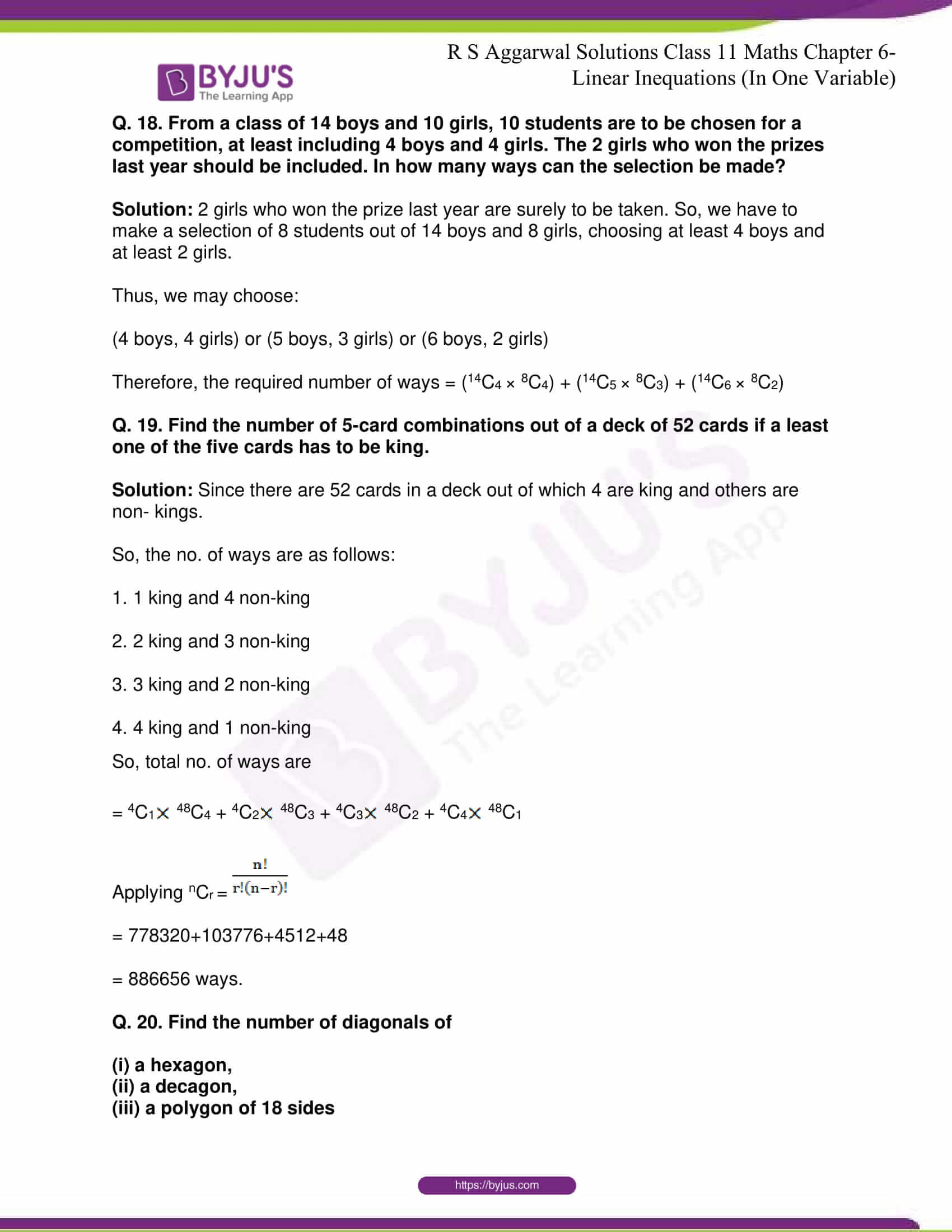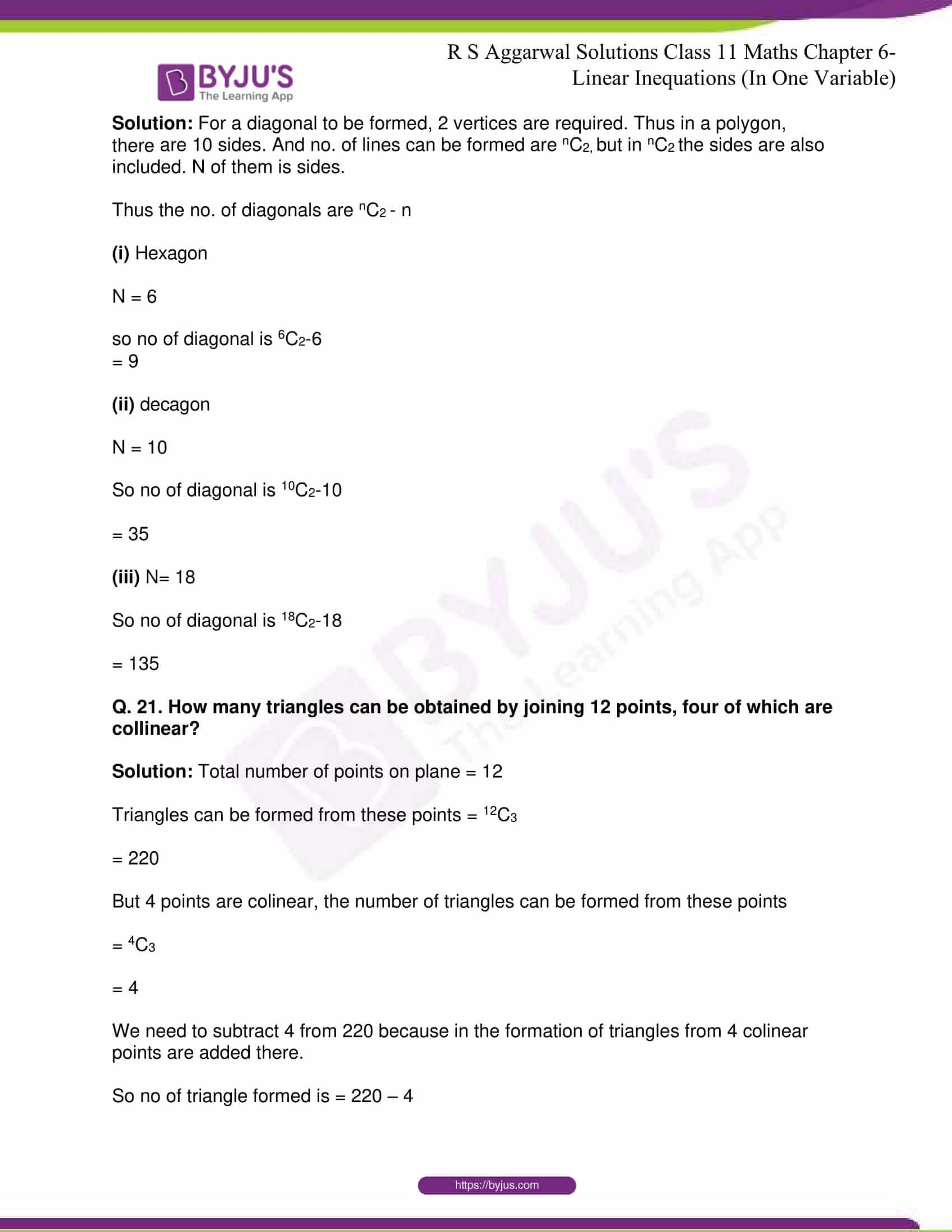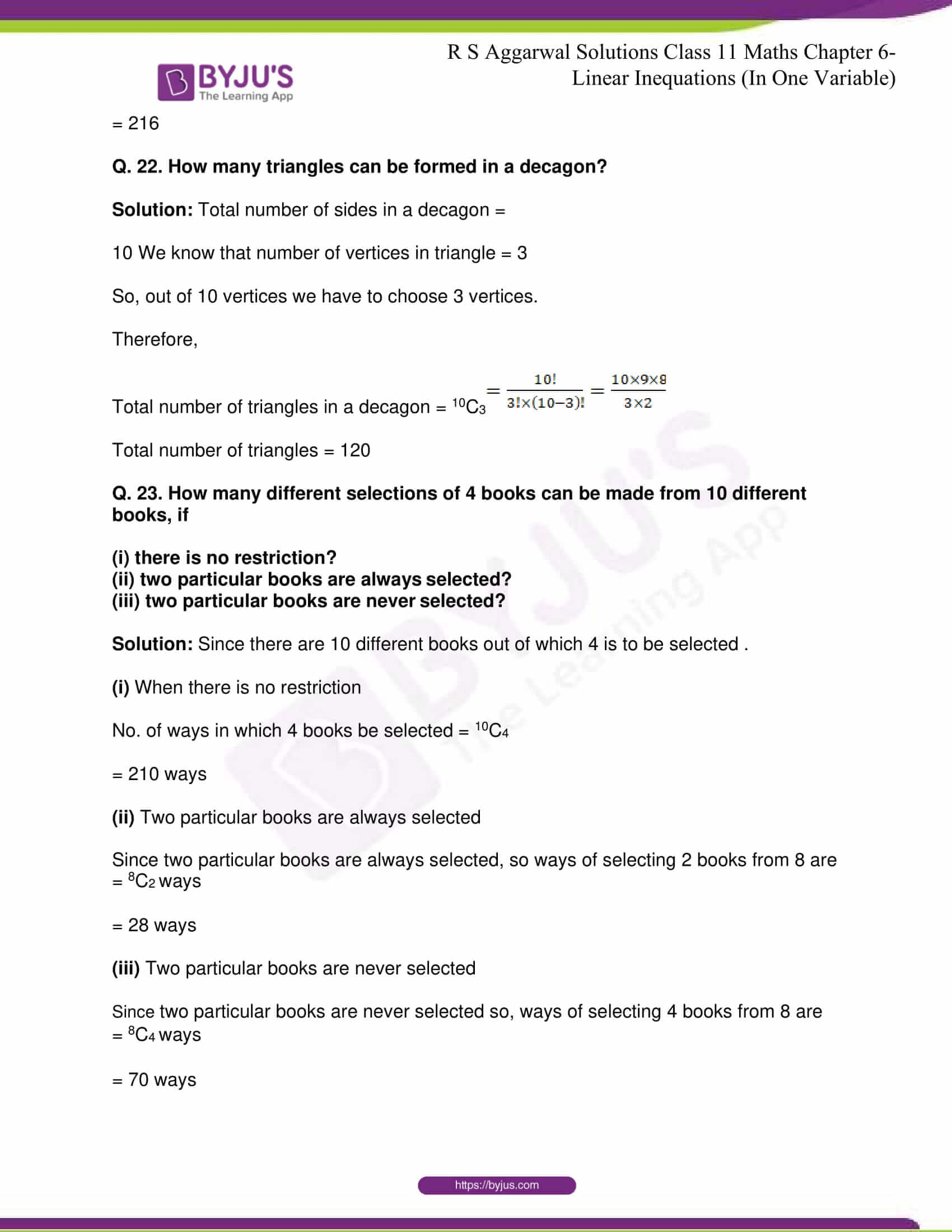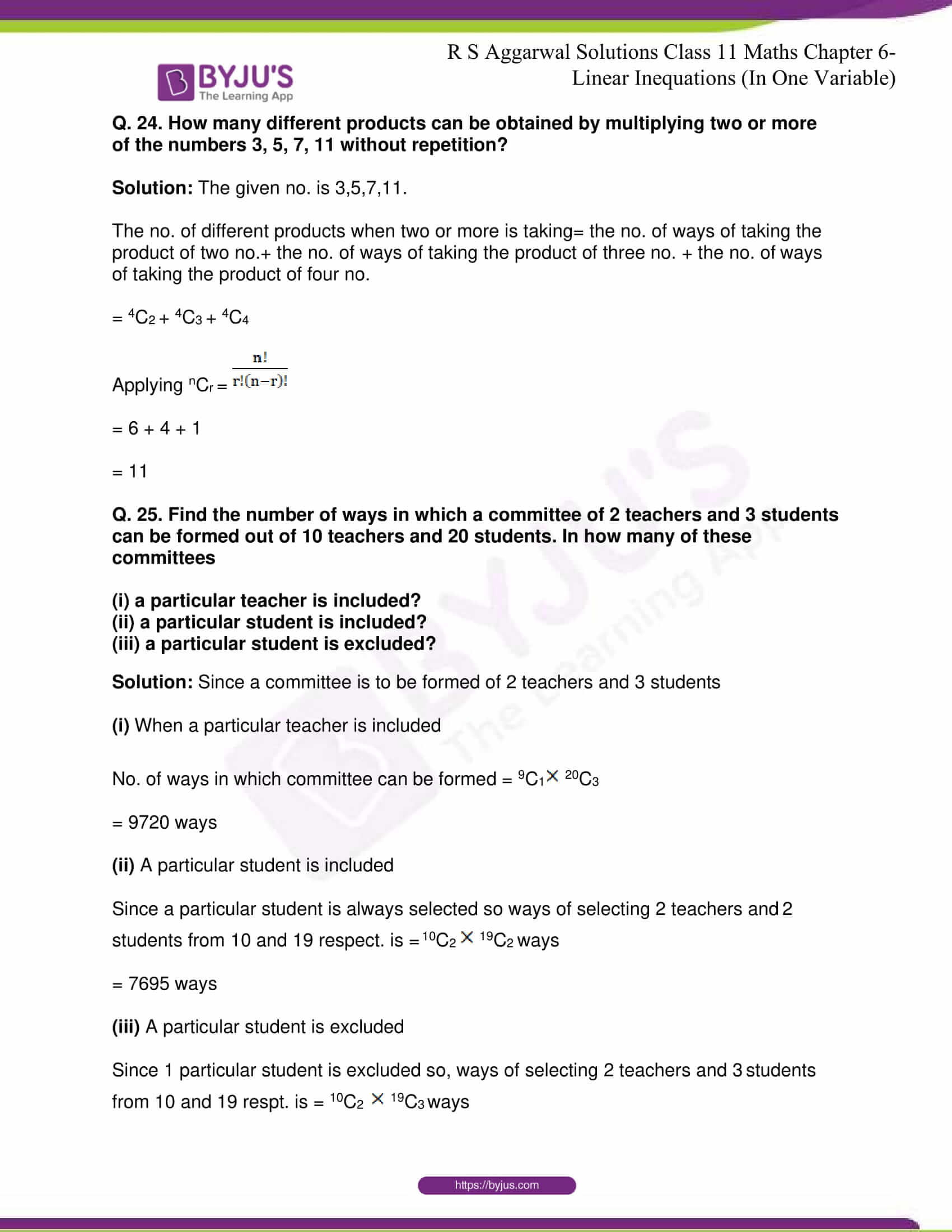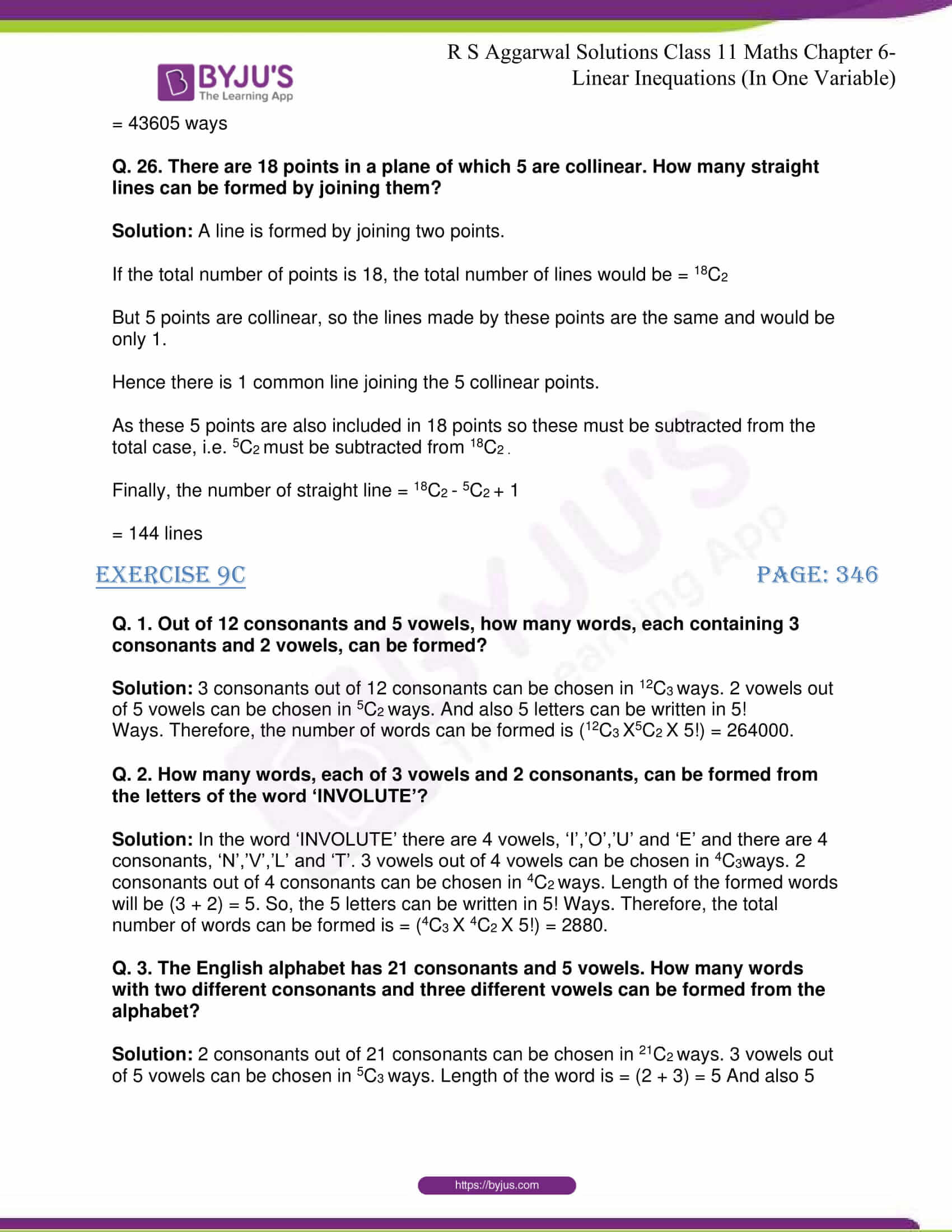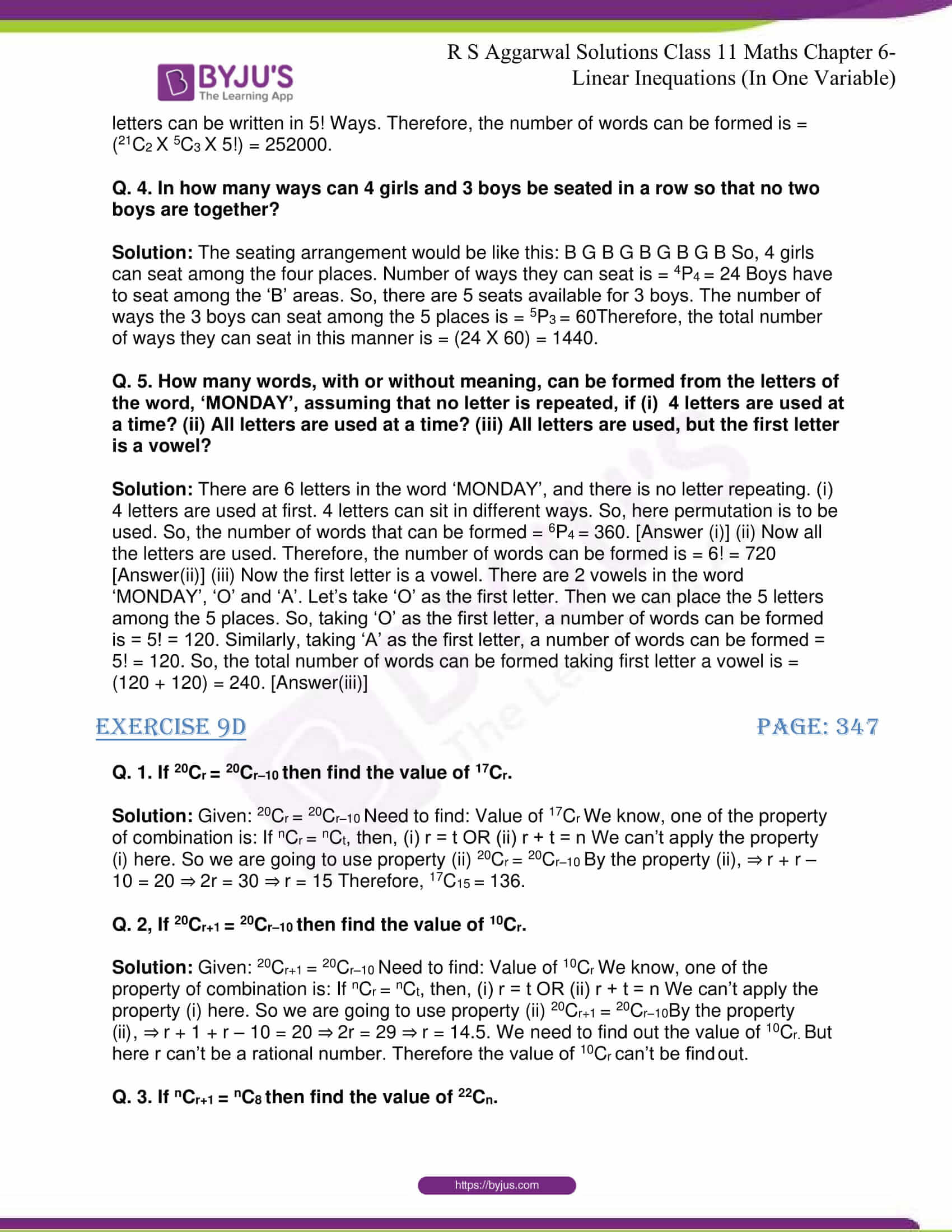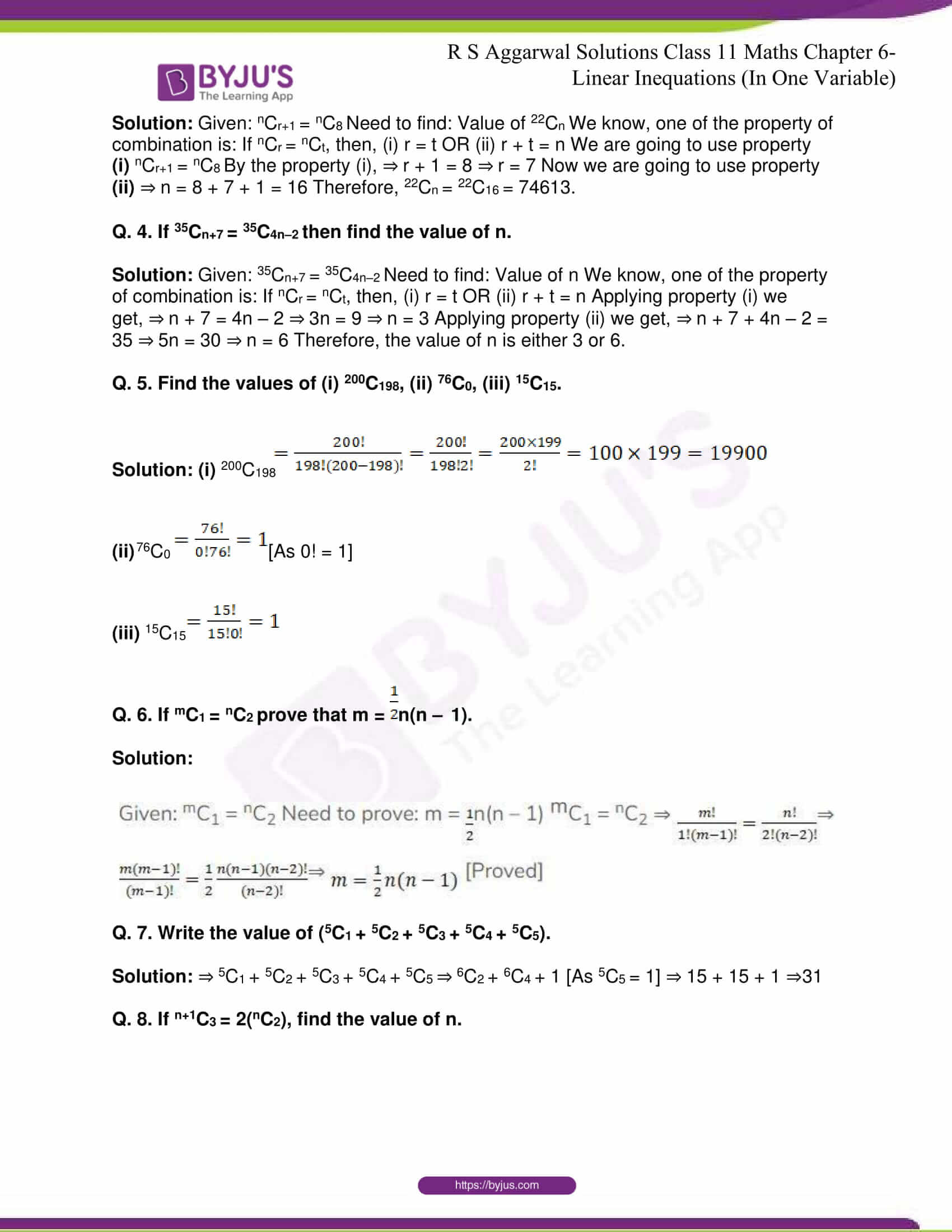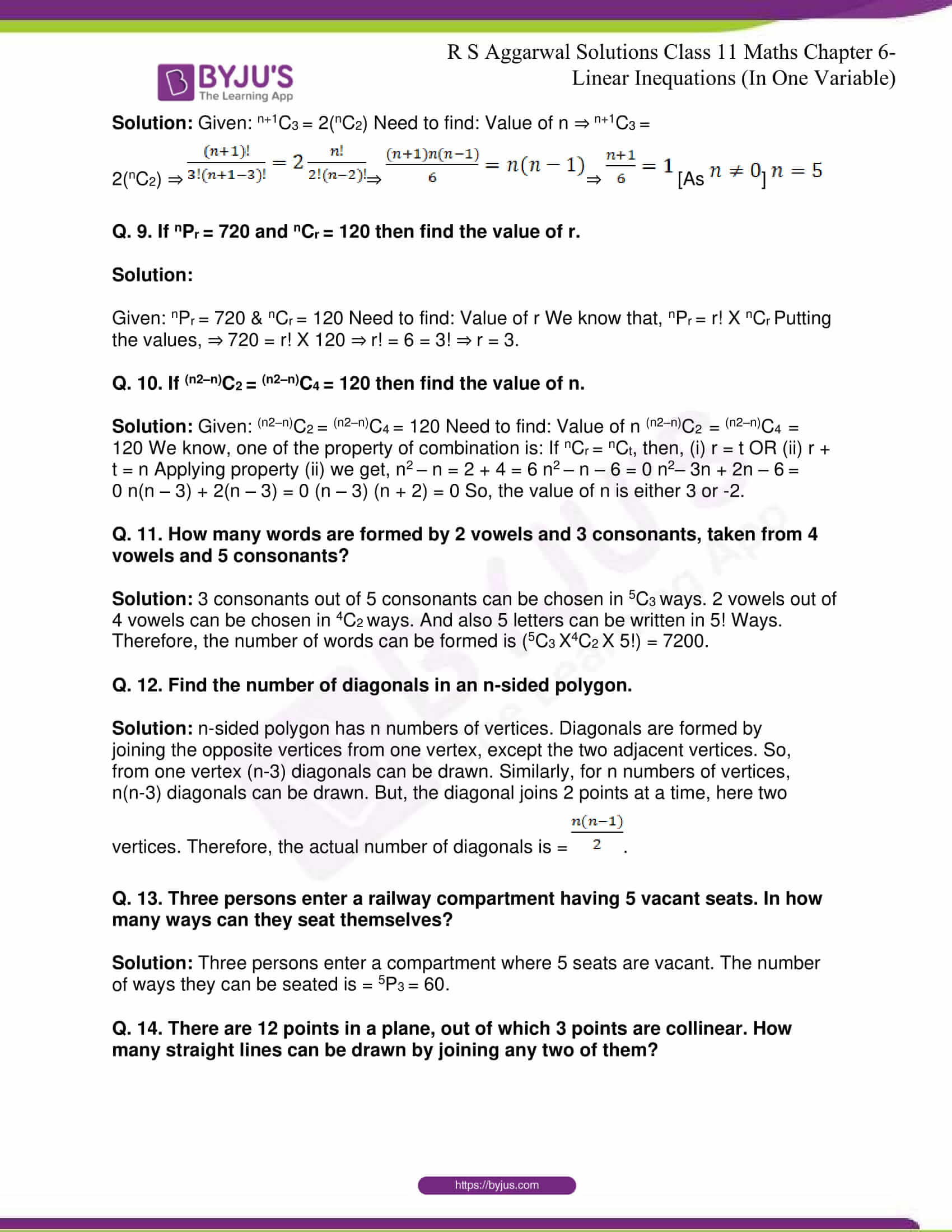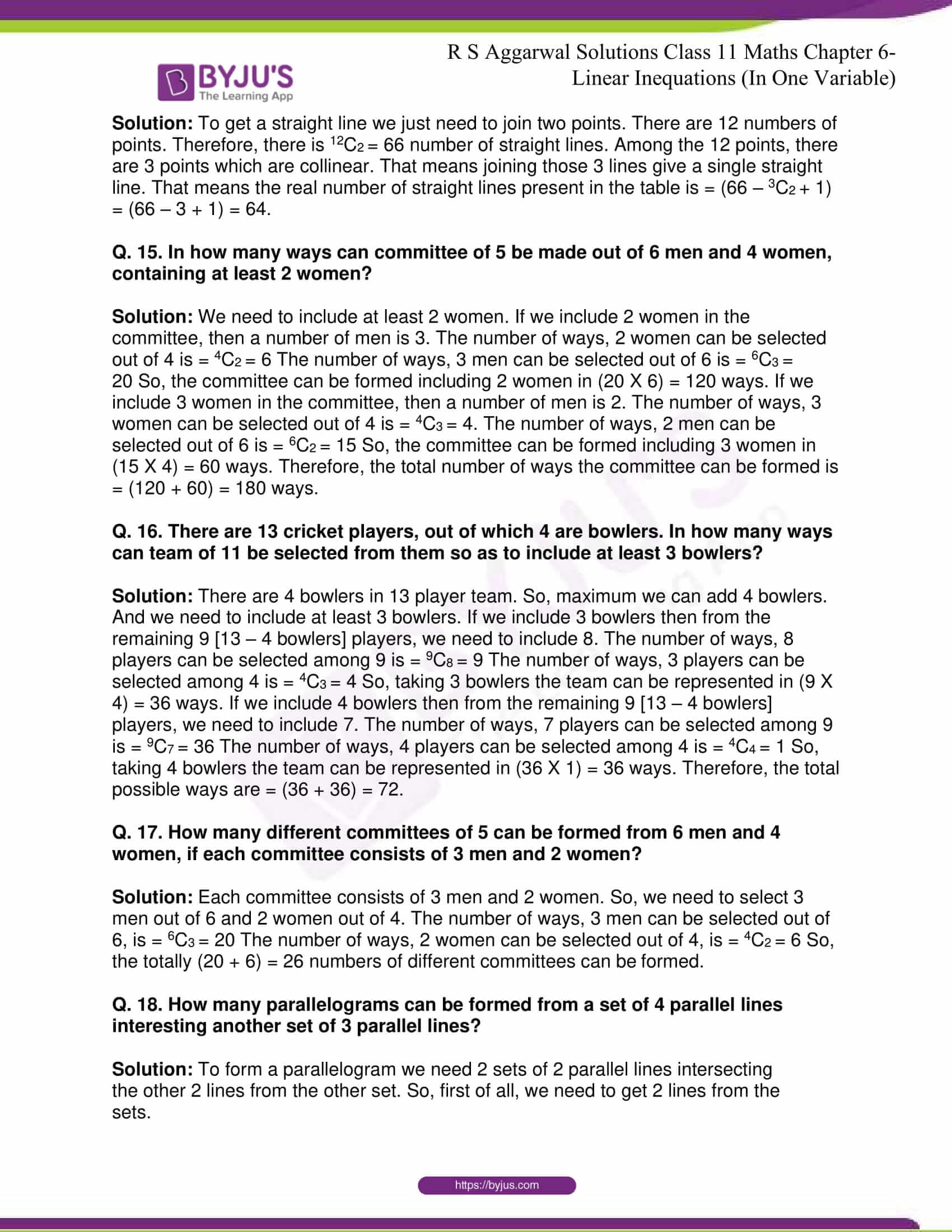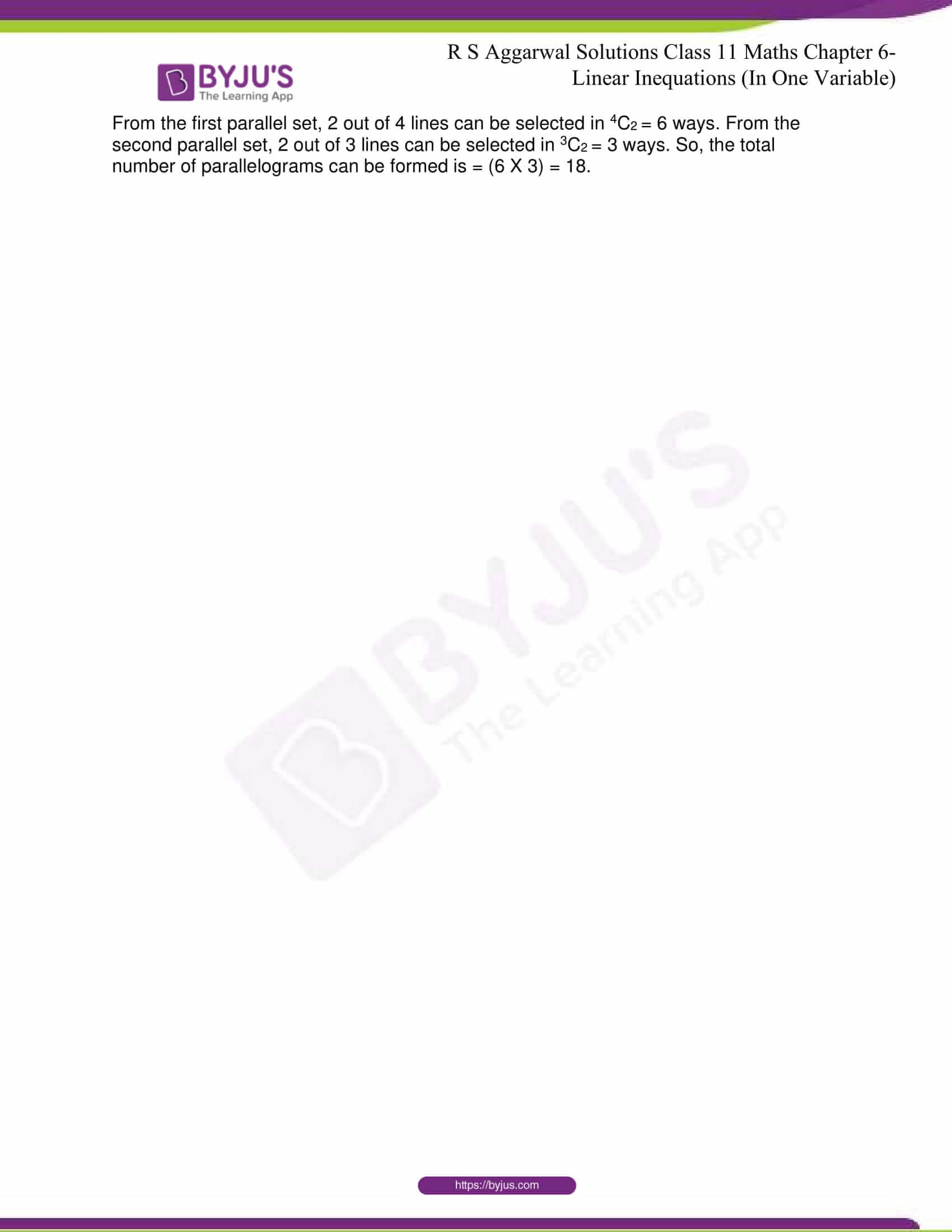## R S Aggarwal Solutions for Class 11 Maths Chapter 9- Combinations

Chapter 9, Combinations, is composed of 4 exercises and the solutions given here contain answers to all the questions present in this exercise. Let us have a look at some of the topics that are being discussed in this chapter.

9.1 Combinations

9.2 Difference Between Permutations and Combinations

9.3 Properties

9.4 Practical Problems on Combinations

9.5 Problems on Permutations and Combinations

## R S Aggarwal Solutions for Class 11 Maths Chapter 9 – Combinations

Each of the different groups or selections that can be made out of a given number of things by taking some or all of them at a time, irrespective of their arrangements, is called a combination. Read and learn Chapter 9 of R S Aggarwal textbook to familiarize with the concepts related to Combinations. Learn the R S Aggarwal Solutions for Class 11 effectively to score high in the examination.